Date: 27-07-2019
Author: Achyuthuni Sri Harsha

## Introduction

Classification problems are an important category of problems in analytics in which the response variable $Y$ takes a discrete value. For example, a classification goal is to analyse what sorts of people were likely to survive the titanic.
The data used in this blog is taken from a very famous problem in Kaggle. Please visit the link for the data description and problem statement.

In particular, in this blog I want to use Logistic regression for the analysis.

The summary statistics for the data is:

##  Survived Pclass      Sex           Age            SibSp
##  I:340    1:214   female:312   Min.   : 0.42   Min.   :0.0000
##  O:549    2:184   male  :577   1st Qu.:22.00   1st Qu.:0.0000
##           3:491                Median :28.05   Median :0.0000
##                                Mean   :29.59   Mean   :0.5242
##                                3rd Qu.:36.00   3rd Qu.:1.0000
##                                Max.   :80.00   Max.   :8.0000
##      Parch             Fare         Embarked
##  Min.   :0.0000   Min.   :  0.000   C:168
##  1st Qu.:0.0000   1st Qu.:  7.896   Q: 77
##  Median :0.0000   Median : 14.454   S:644
##  Mean   :0.3825   Mean   : 32.097
##  3rd Qu.:0.0000   3rd Qu.: 31.000
##  Max.   :6.0000   Max.   :512.329

## Data Cleaning and EDA

Zero and Near Zero Variance features do not explain any variance in the predictor variable.

nearZeroVar(raw_df, saveMetrics= TRUE)

There are no near zero or zero variance columns

Similarly I can check for linearly dependent columns among the continuous variables.

feature_map <- unlist(lapply(raw_df, is.numeric))
findLinearCombos((raw_df[,feature_map]))
## $linearCombos ## list() ## ##$remove
## NULL

There are no linearly dependent columns.

### Uni-variate analysis

Now, I want to do some basic EDA on each column. On each continuous column, I want to visually check the following:
1. Variation in the column
2. Its distribution
3. Any outliers
4. q-q plot with normal distribution

cont_univ_df <- raw_df %>% select_if(is.numeric) %>% mutate(row_no = as.numeric(rownames(raw_df)))

for(column in colnames(cont_univ_df[-ncol(cont_univ_df)])){
p1 <- ggplot(cont_univ_df, aes_string(x='row_no', y= column)) +
geom_point(show.legend = FALSE) +
labs(x = 'Univariate plot', y=column) +
ggtitle(column) +
theme_minimal()

# Cumulative plot
legendcols <- c("Normal distribution"="darkred","Density"="darkBlue","Histogram"="lightBlue")
p2 <- ggplot(cont_univ_df,aes_string(x = column)) +
geom_histogram(aes(y=..density.., fill ="Histogram"), bins = 50) +
stat_function(fun = dnorm, aes(color="Normal distribution"),  size = 1,
args = list(mean = mean(cont_univ_df[[column]]),
sd = sd(cont_univ_df[[column]]))) +
geom_density(aes(y=..density.., color="Density"),  size = 1)+
scale_colour_manual(name="Distribution",values=legendcols) +
scale_fill_manual(name="Bar",values=legendcols) +
theme_minimal() + theme(legend.position="none")

p3 <- ggplot(cont_univ_df %>% mutate(constant = column),
aes_string(x="constant", y= column, group = 123)) +
geom_boxplot() +
labs(y=column) +
theme_minimal()

p4 <- ggplot(cont_univ_df,aes_string(sample = column)) +
stat_qq() + stat_qq_line() +
theme_minimal()
grid.arrange(p1, p2, p3, p4, nrow=2)
}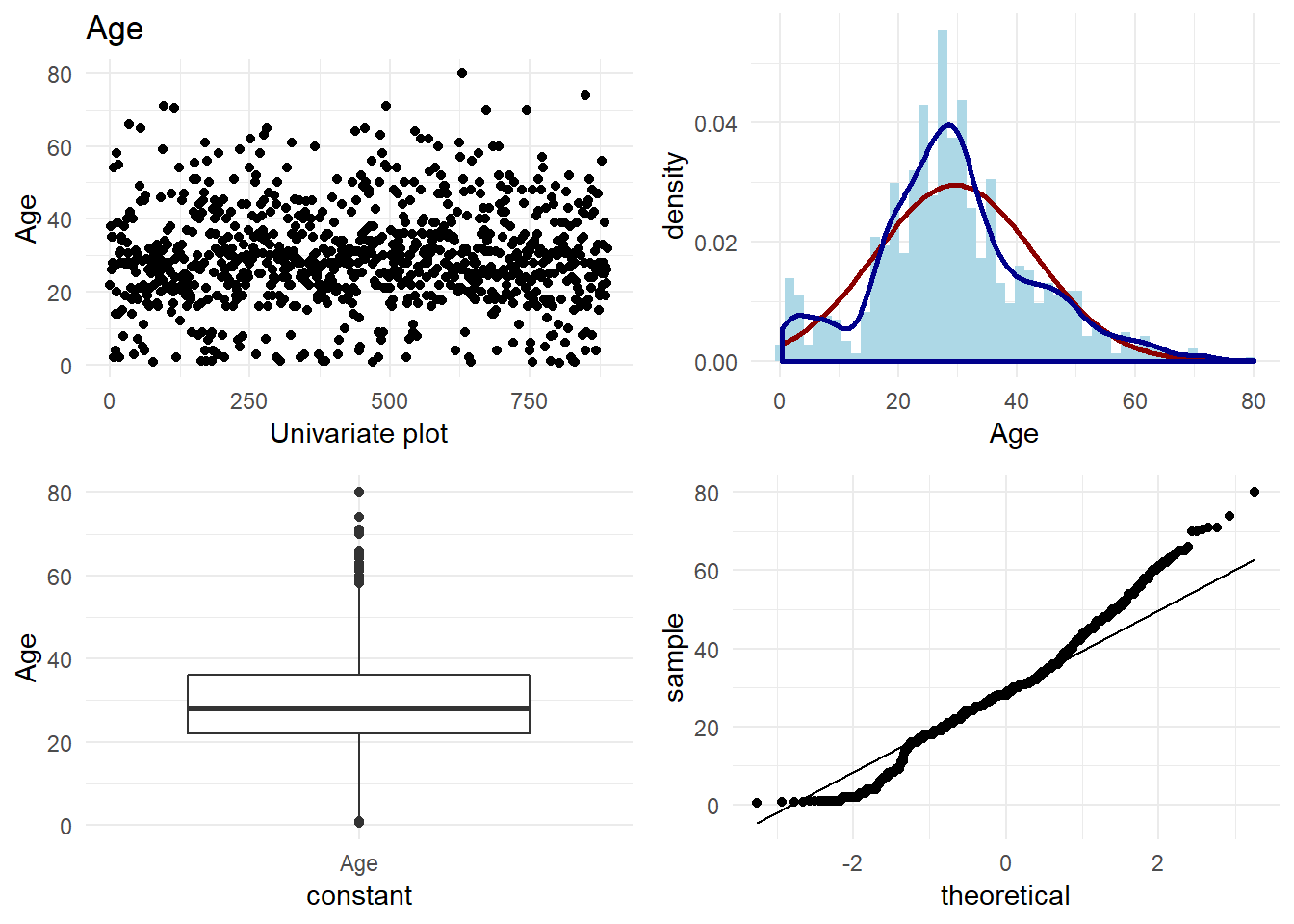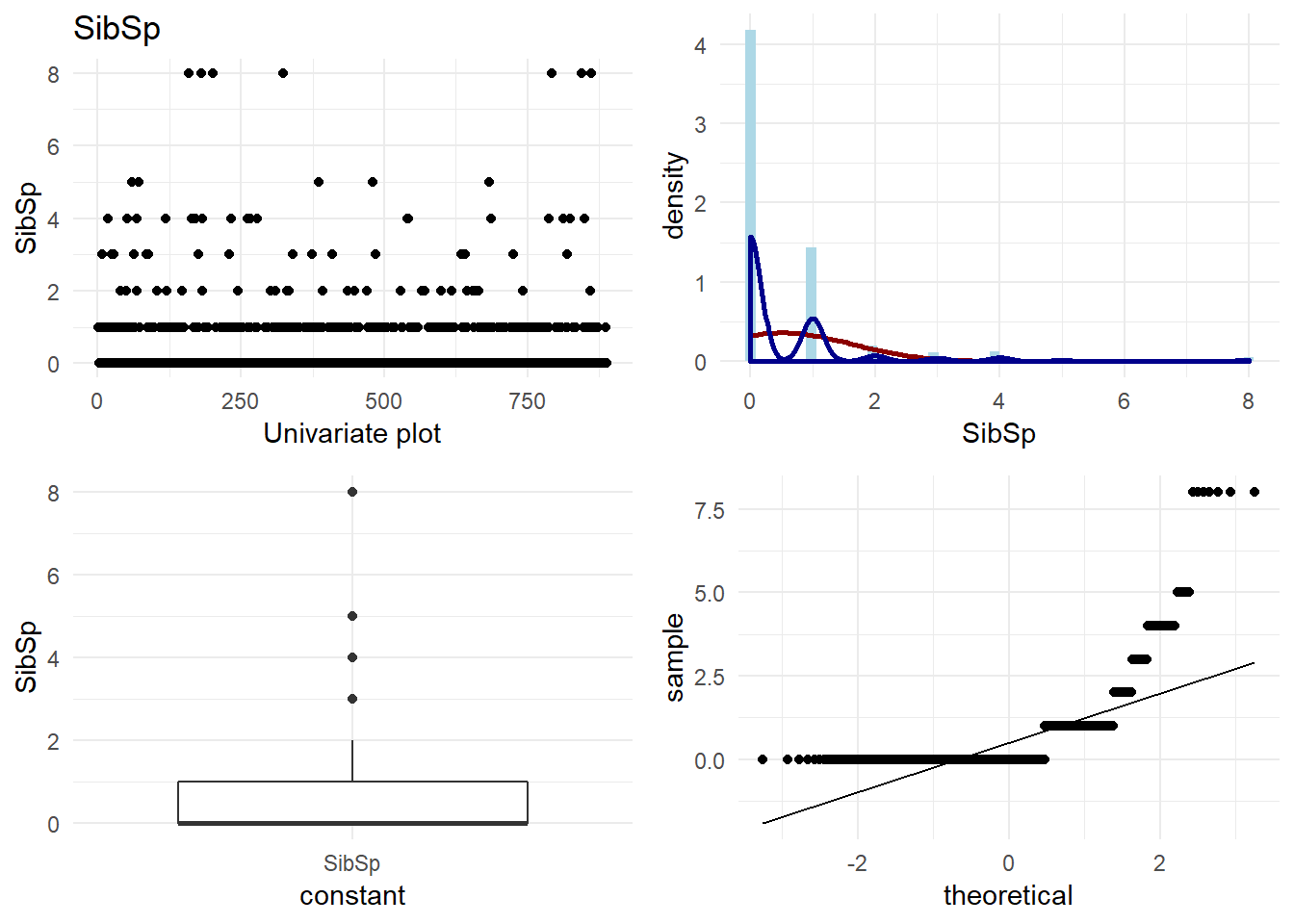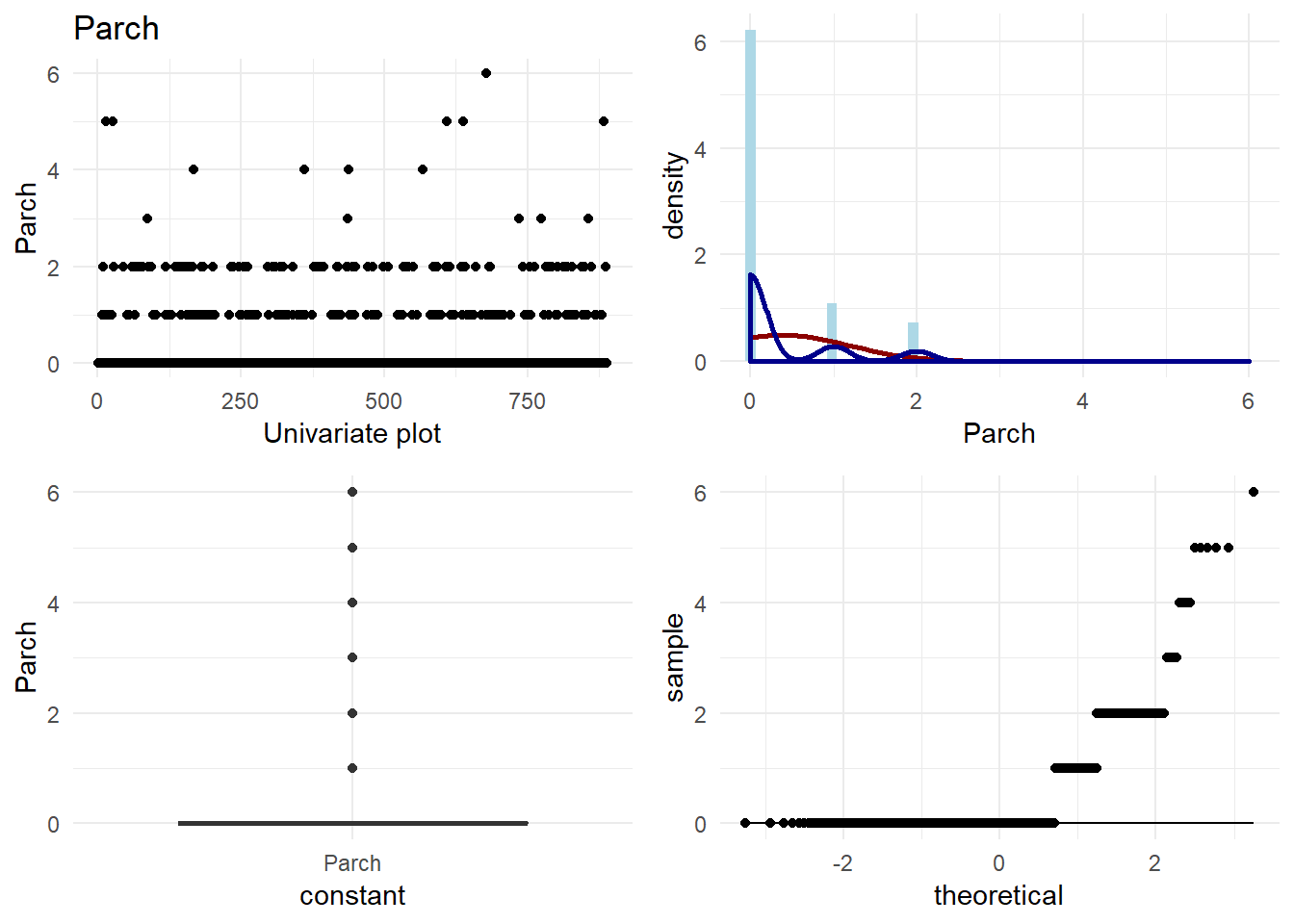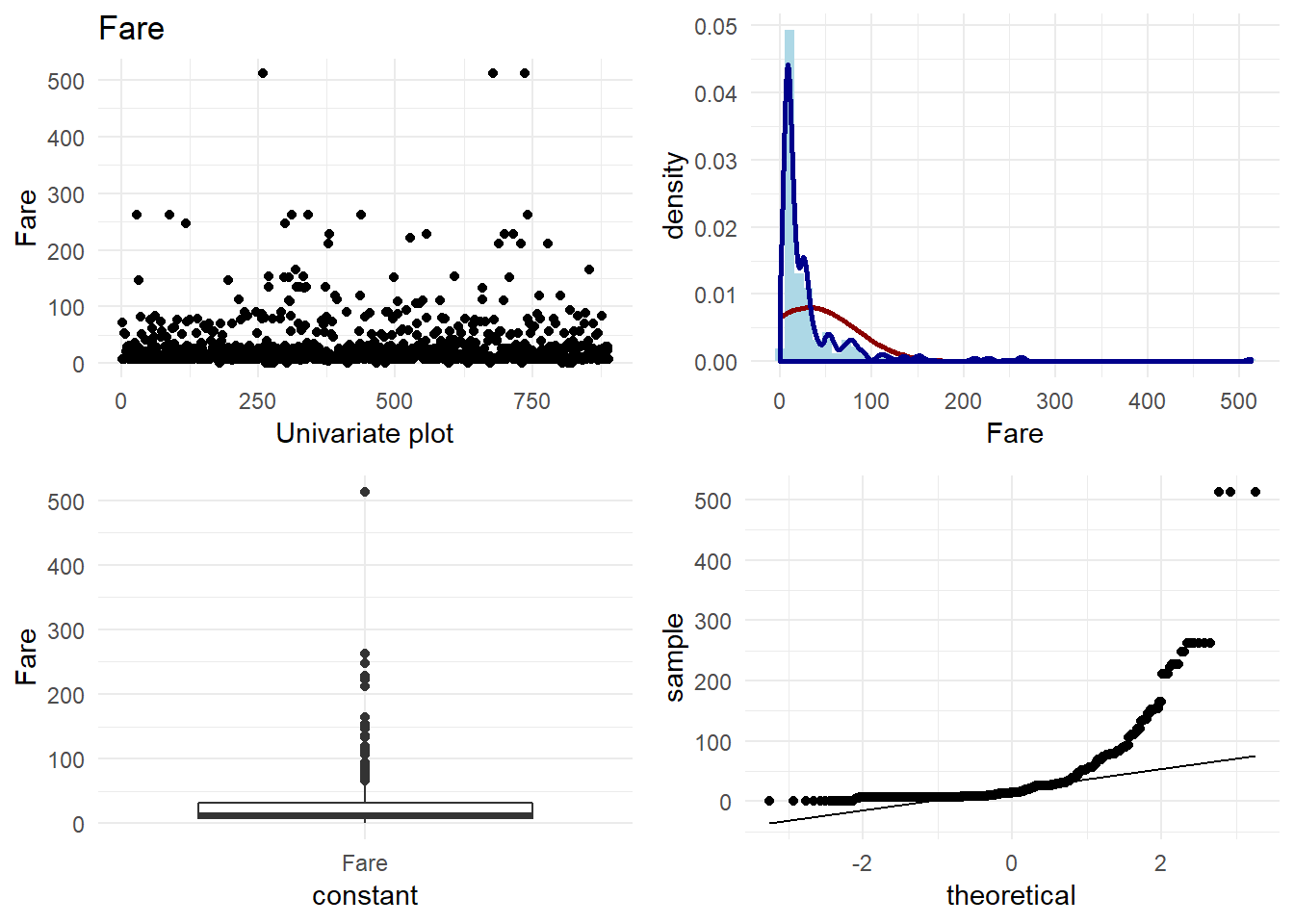For categorical variables, I want to look at the frequencies.

univ_cat_df <- raw_df %>% select_if(function(col) {is.factor(col) | is.character(col)})
for(column in colnames(univ_cat_df)){
plot(ggplot(univ_cat_df,aes_string(column)) +
geom_bar() + coord_flip() +
ggtitle(column) +
theme_minimal())
}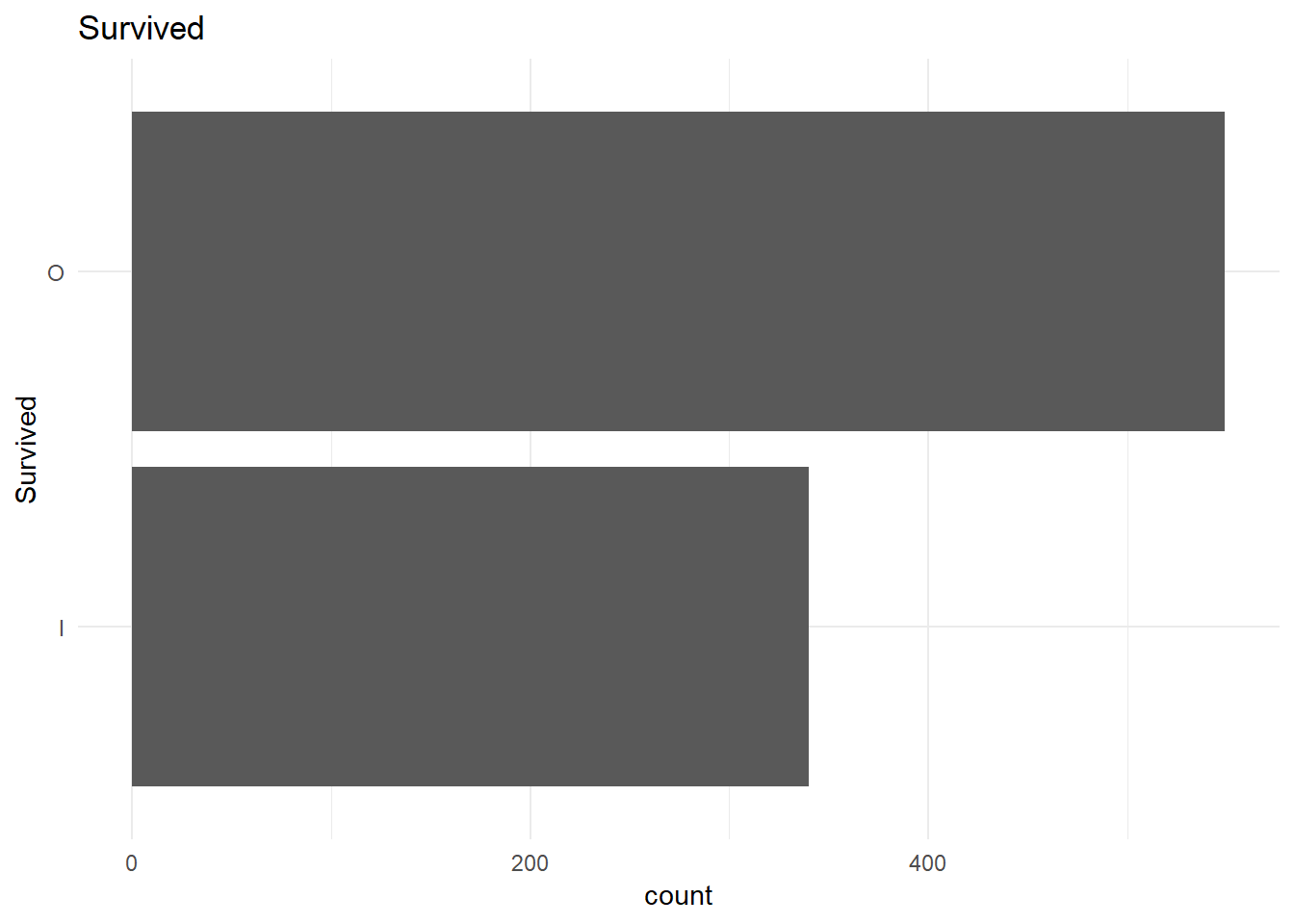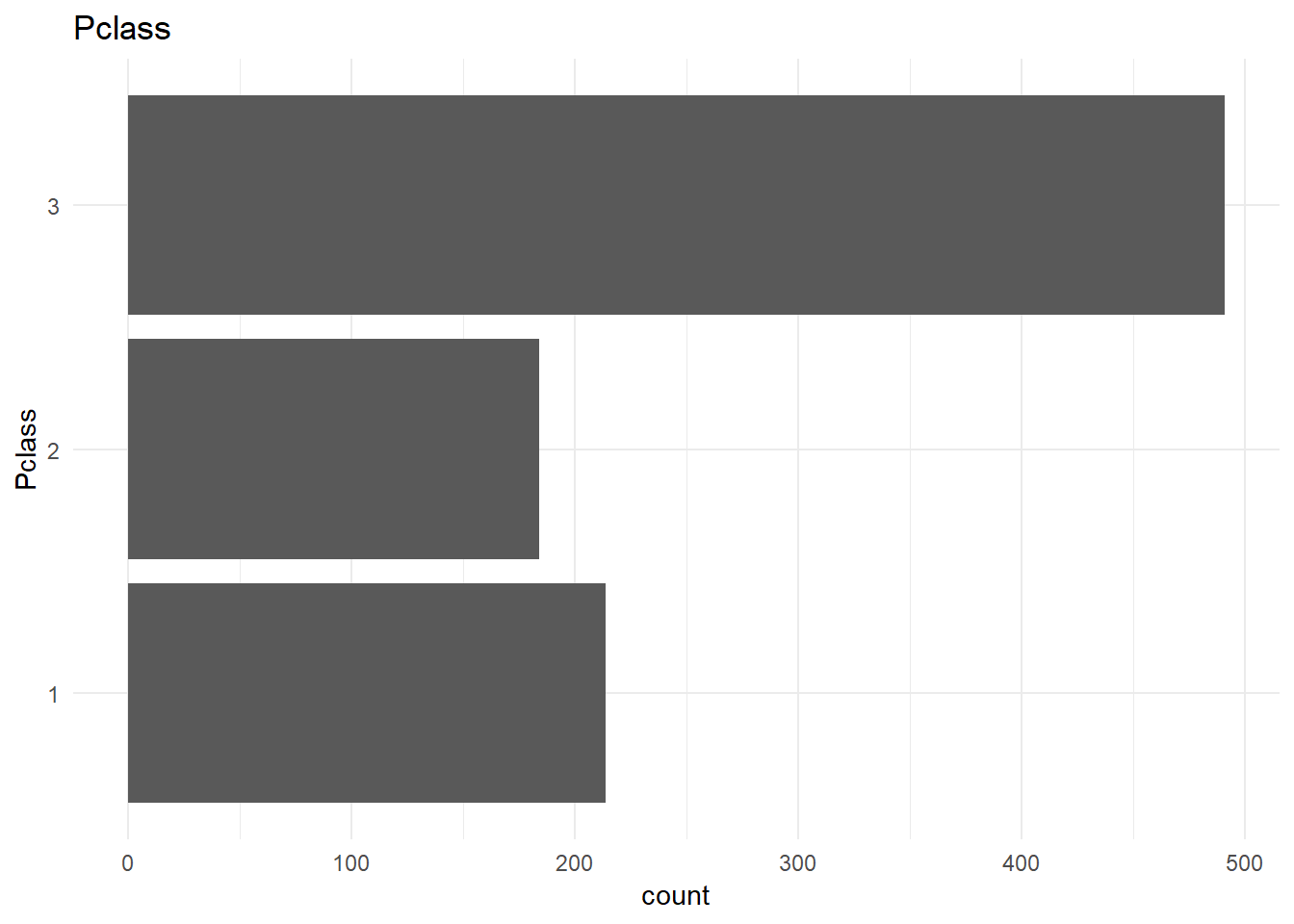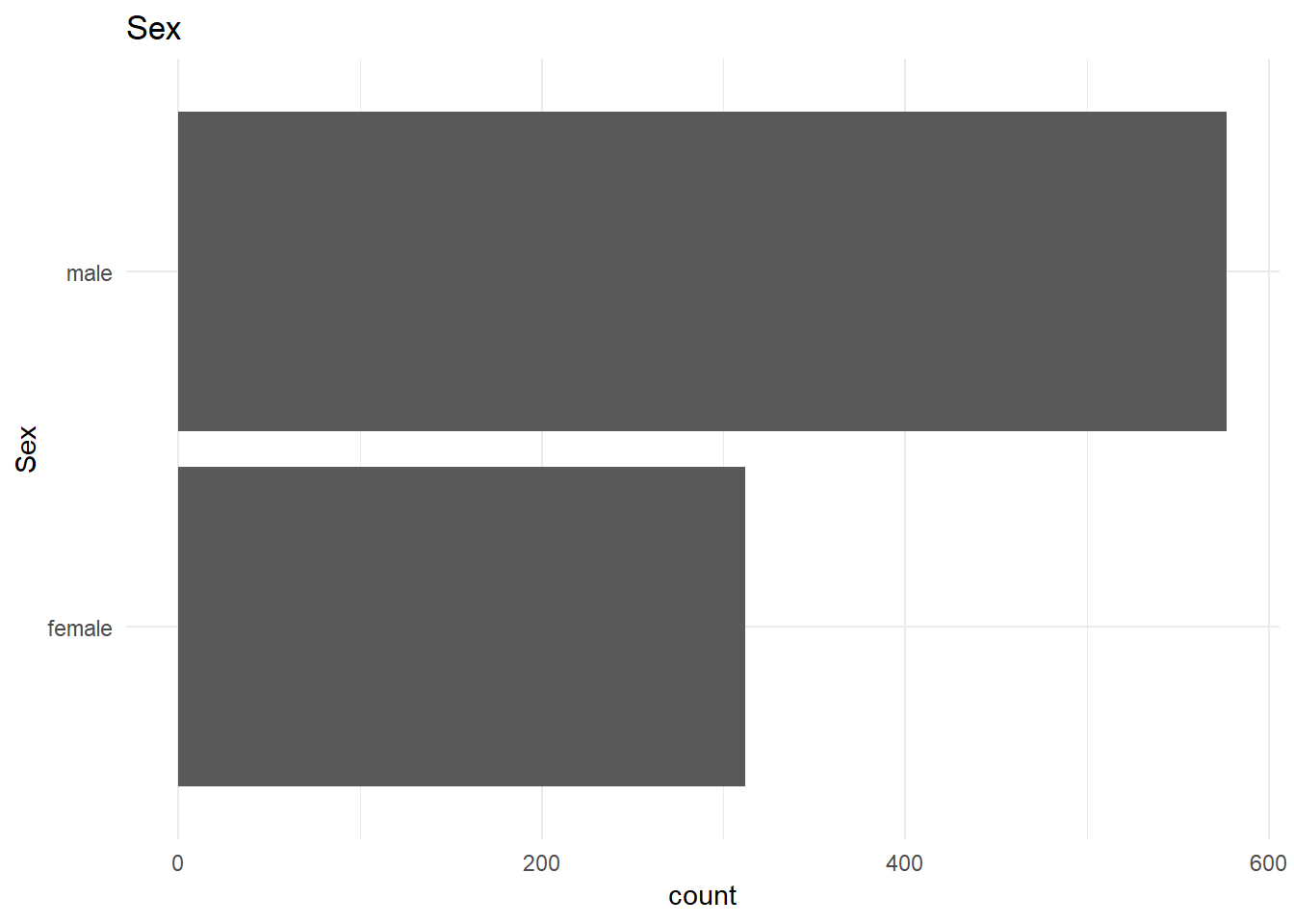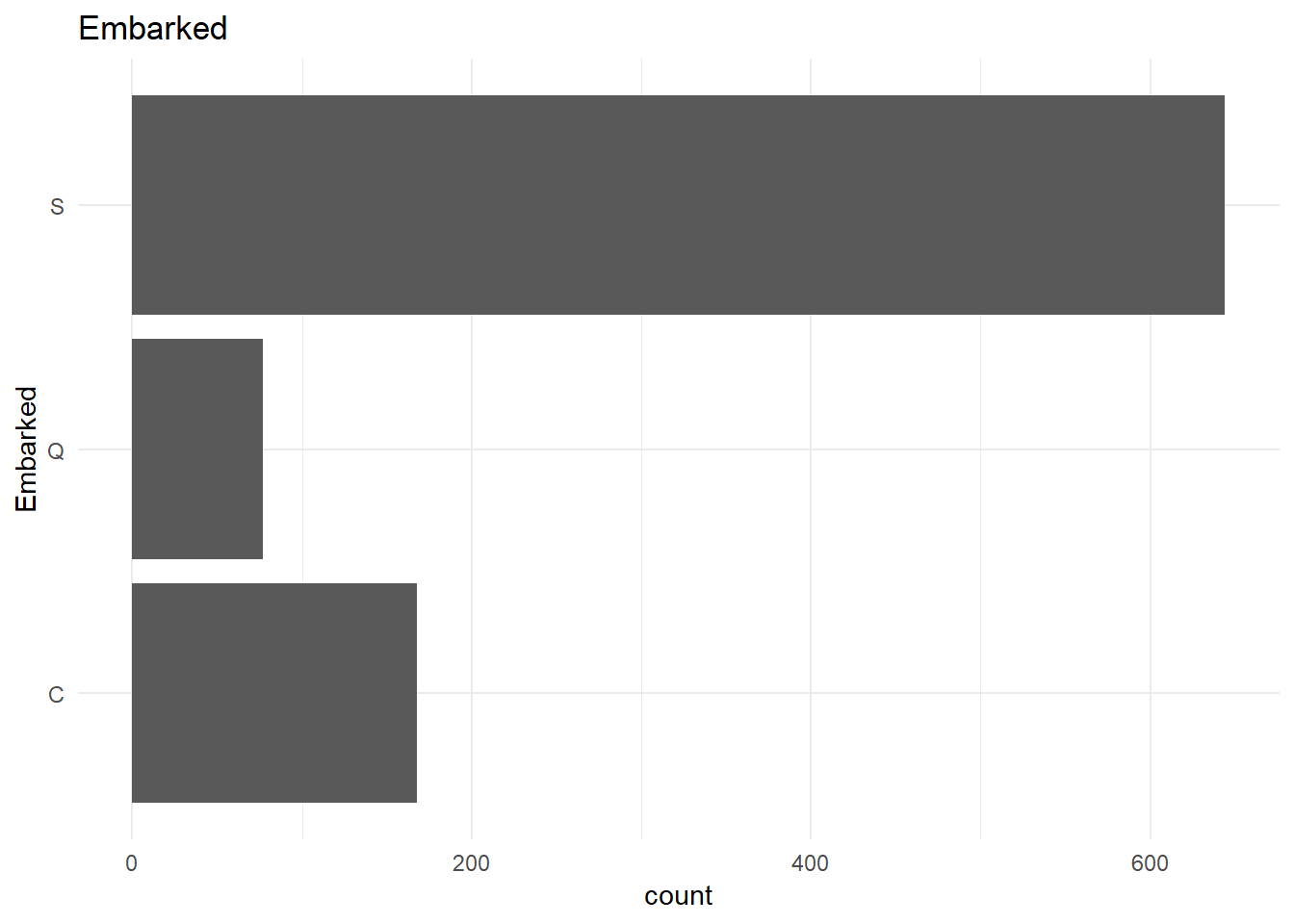From the above plot I infer that the data is unbalanced. But it might not be a problem as the unbalance ratio is less than 2:1.

### Bi variate analysis

I want to understand the relationship of each continuous variable with the $y$ variable. I will achieve that by doing the following:
1. Plot box plot for each of the variables to do a visual comparison between the groups
2. Plot the explanatory variable distribution for both the variables to understand the variability uniquely explained (The non-intersecting part of the blue and the pink is the variation explained by the variable)
3. Predict using Logistic regression using the variable alone to observe the decrease in deviation/AIC
4. Plot Lorenz curve to compute Gini coefficient if applicable (high gini coefficient means that high inequality is caused by the column, which means more explain-ability)

library(gglorenz)
library(ineq)
plot_bivariate_cont <- function(raw_data, pred_column_name){
bi_var_df <- raw_df %>% select_if(is.numeric)
for(column in colnames(bi_var_df)){
p1 <- ggplot(raw_df,
aes_string(x = pred_column_name, y= column, group = pred_column_name)) +
geom_boxplot() +
labs(y=column) +
ggtitle(column) +
theme_minimal()

p2 <- ggplot(raw_df, aes_string(x=column, fill=pred_column_name)) +
geom_histogram(alpha=0.5, position="identity") +
theme_minimal()+ theme(legend.position="bottom")

grid.arrange(p1, p2, nrow=1, widths = c(1,2))

trainList_bi <- createDataPartition(y = unlist(raw_df[pred_column_name]), times = 1,p = 0.8, list = FALSE)
dfTest_bi <- raw_df[-trainList_bi,]
dfTrain_bi <- raw_df[trainList_bi,]
form_2 = as.formula(paste0(pred_column_name,' ~ ',column))
set.seed(1234)
objControl <- trainControl(method = "none",
summaryFunction = twoClassSummary,
# sampling = 'smote',
classProbs = TRUE)

cont_loop_caret_model <- train(form_2, data = dfTrain_bi,
method = 'glm',
trControl = objControl,
metric = "ROC"
)
print(summary(cont_loop_caret_model))
if(sum(raw_df[column]<0) == 0){
plot(ggplot(raw_df, aes_string(column)) +
gglorenz::stat_lorenz(color = "red") +
geom_abline(intercept = 0, slope = 1, color = 'blue') +
theme_minimal())
print(paste0('Gini coefficient = ', Gini(unlist(raw_df[column]))))
}
print(strrep("-",100))
}
}
plot_bivariate_cont(raw_df, pred_column_name = 'Survived')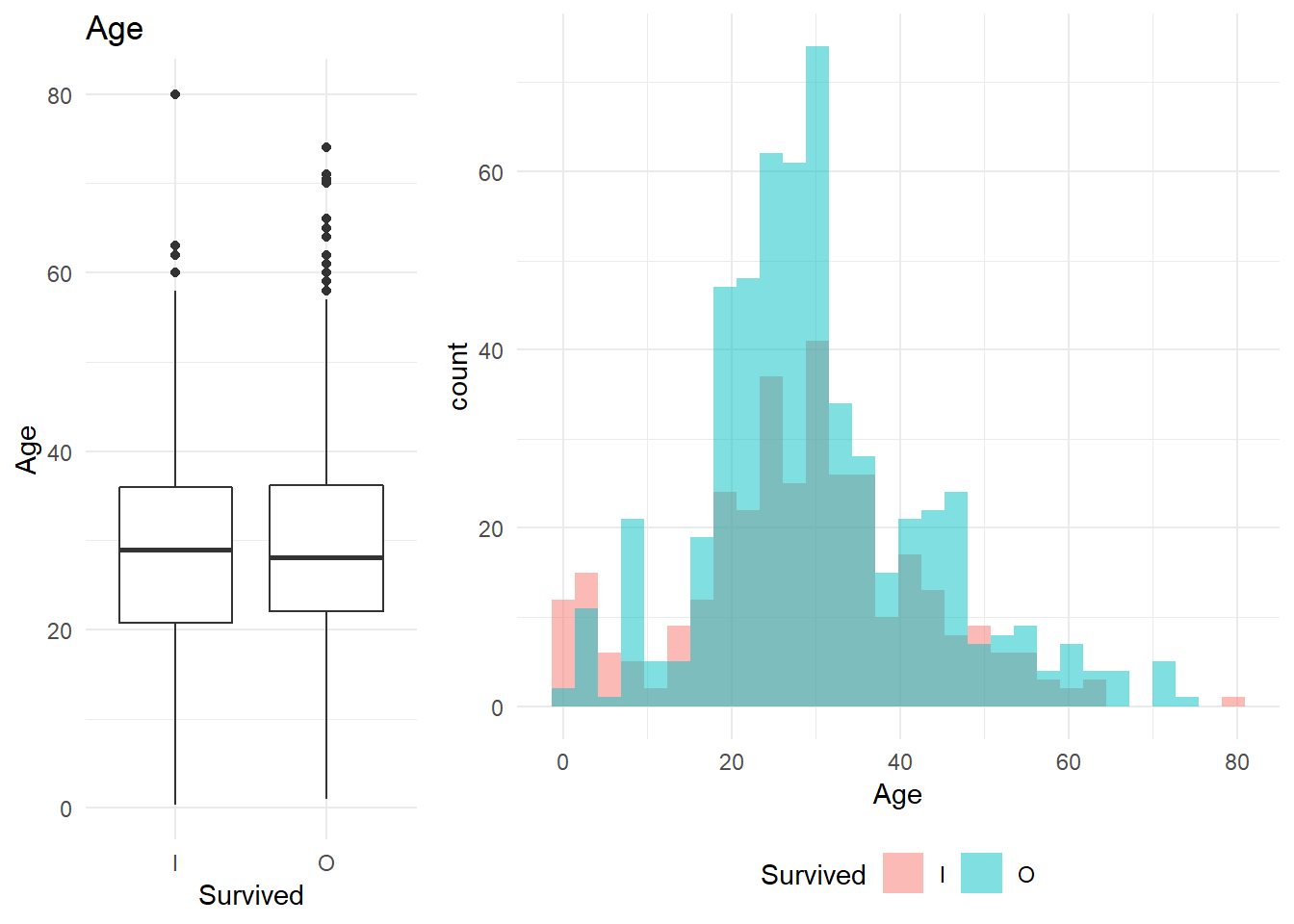##
## Call:
## NULL
##
## Deviance Residuals:
##     Min       1Q   Median       3Q      Max
## -1.5682  -1.3694   0.9455   0.9906   1.0696
##
## Coefficients:
##             Estimate Std. Error z value Pr(>|z|)
## (Intercept) 0.251045   0.184309   1.362    0.173
## Age         0.007909   0.005791   1.366    0.172
##
## (Dispersion parameter for binomial family taken to be 1)
##
##     Null deviance: 947.02  on 711  degrees of freedom
## Residual deviance: 945.15  on 710  degrees of freedom
## AIC: 949.15
##
## Number of Fisher Scoring iterations: 4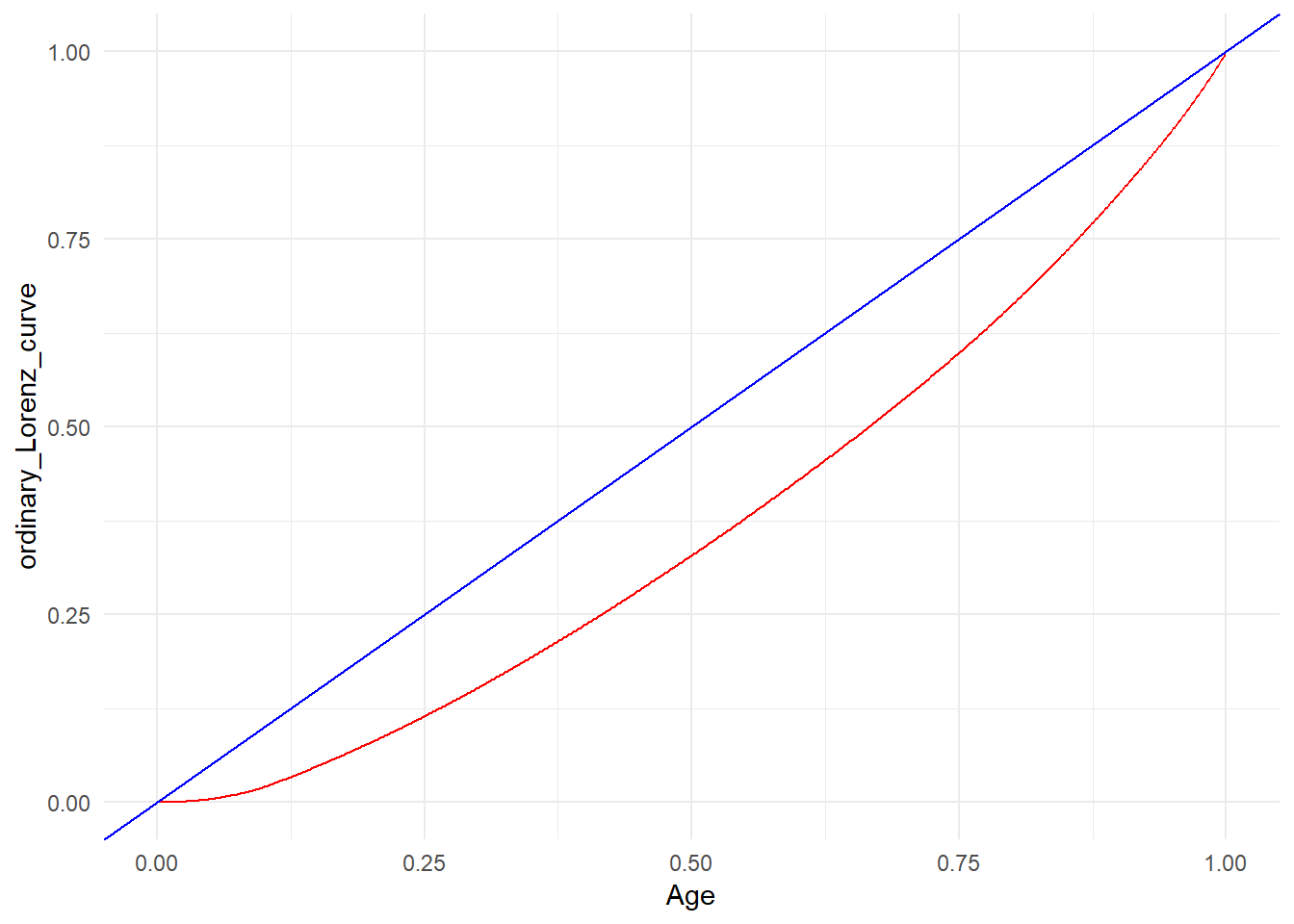##  "Gini coefficient = 0.25155022394829"
##  "----------------------------------------------------------------------------------------------------"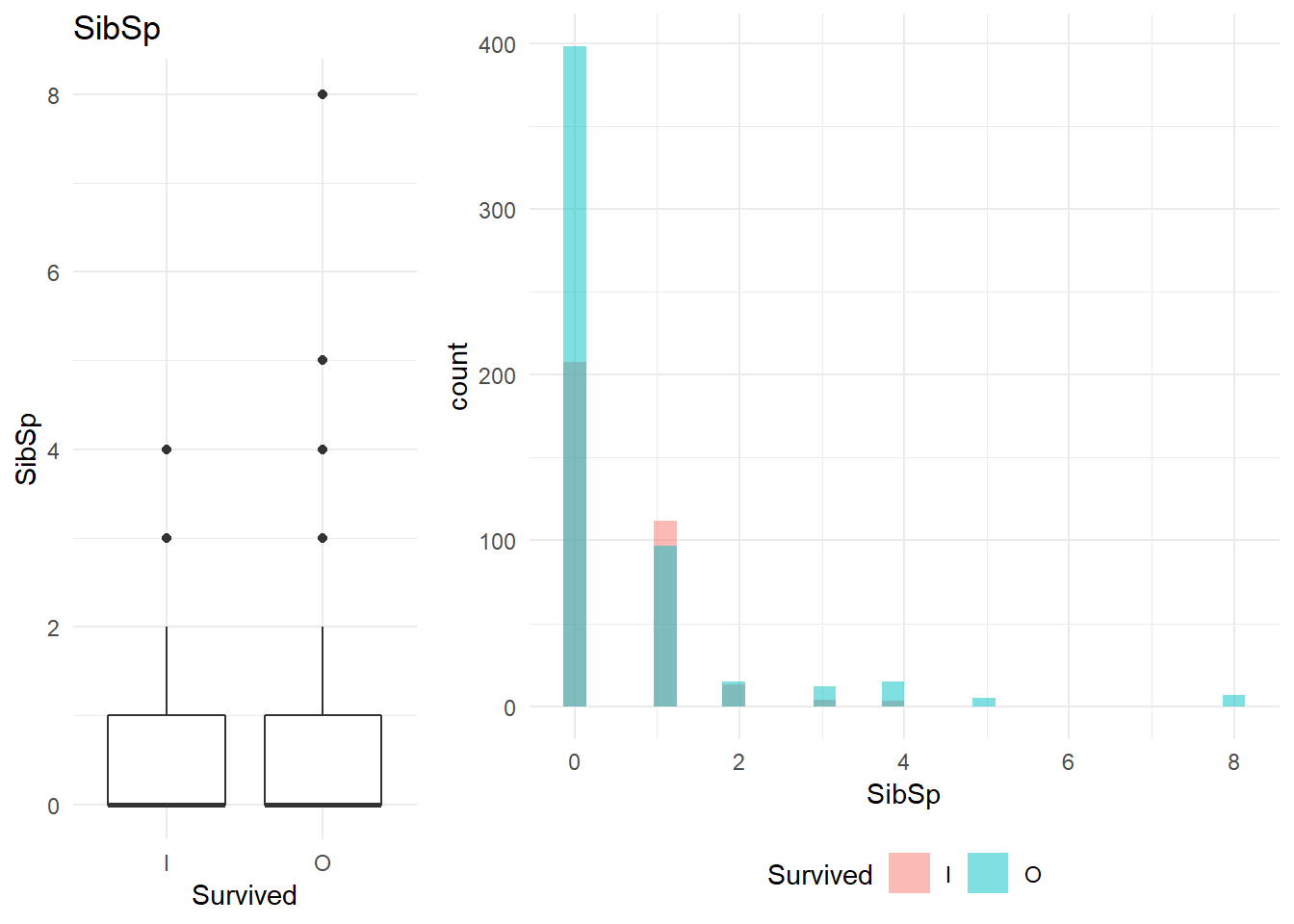##
## Call:
## NULL
##
## Deviance Residuals:
##     Min       1Q   Median       3Q      Max
## -1.4934  -1.3714   0.9685   0.9950   0.9950
##
## Coefficients:
##             Estimate Std. Error z value Pr(>|z|)
## (Intercept)  0.44536    0.08507   5.235 1.65e-07 ***
## SibSp        0.06812    0.07026   0.970    0.332
## ---
## Signif. codes:  0 '***' 0.001 '**' 0.01 '*' 0.05 '.' 0.1 ' ' 1
##
## (Dispersion parameter for binomial family taken to be 1)
##
##     Null deviance: 947.02  on 711  degrees of freedom
## Residual deviance: 946.05  on 710  degrees of freedom
## AIC: 950.05
##
## Number of Fisher Scoring iterations: 4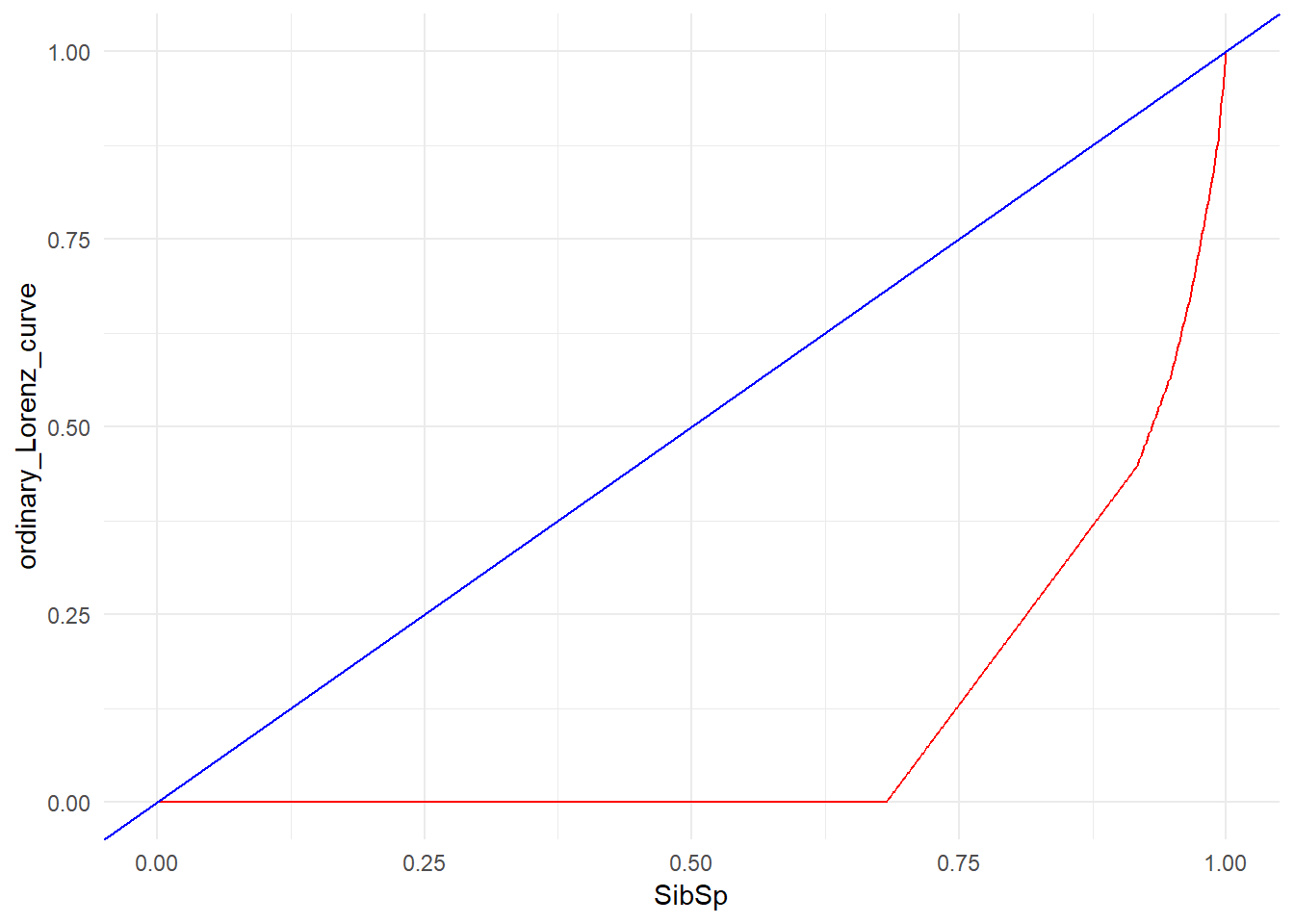##  "Gini coefficient = 0.785475313439897"
##  "----------------------------------------------------------------------------------------------------"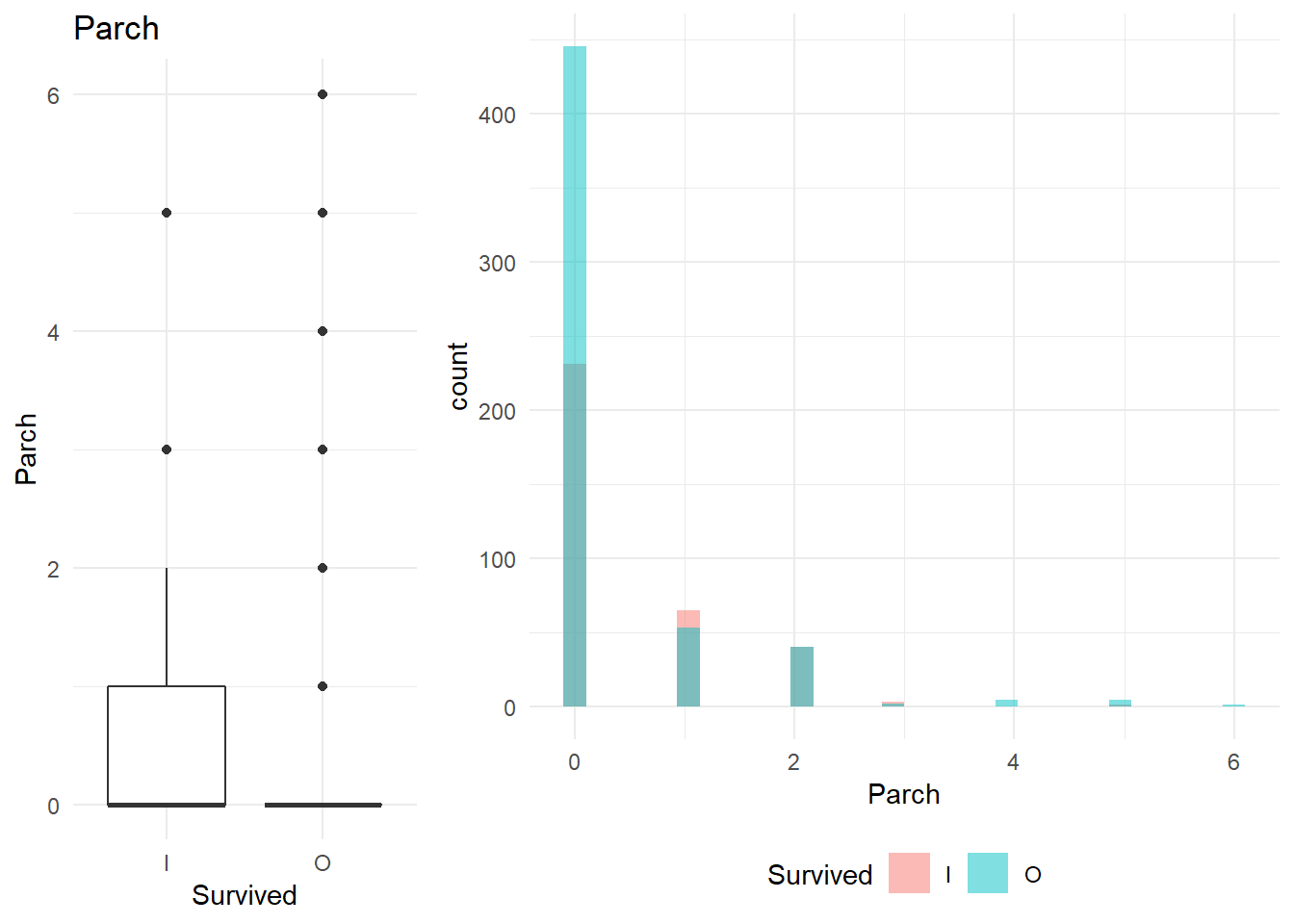##
## Call:
## NULL
##
## Deviance Residuals:
##     Min       1Q   Median       3Q      Max
## -1.4250  -1.4250   0.9486   0.9486   1.4932
##
## Coefficients:
##             Estimate Std. Error z value Pr(>|z|)
## (Intercept)  0.56535    0.08631   6.550 5.75e-11 ***
## Parch       -0.21380    0.09527  -2.244   0.0248 *
## ---
## Signif. codes:  0 '***' 0.001 '**' 0.01 '*' 0.05 '.' 0.1 ' ' 1
##
## (Dispersion parameter for binomial family taken to be 1)
##
##     Null deviance: 947.02  on 711  degrees of freedom
## Residual deviance: 941.94  on 710  degrees of freedom
## AIC: 945.94
##
## Number of Fisher Scoring iterations: 4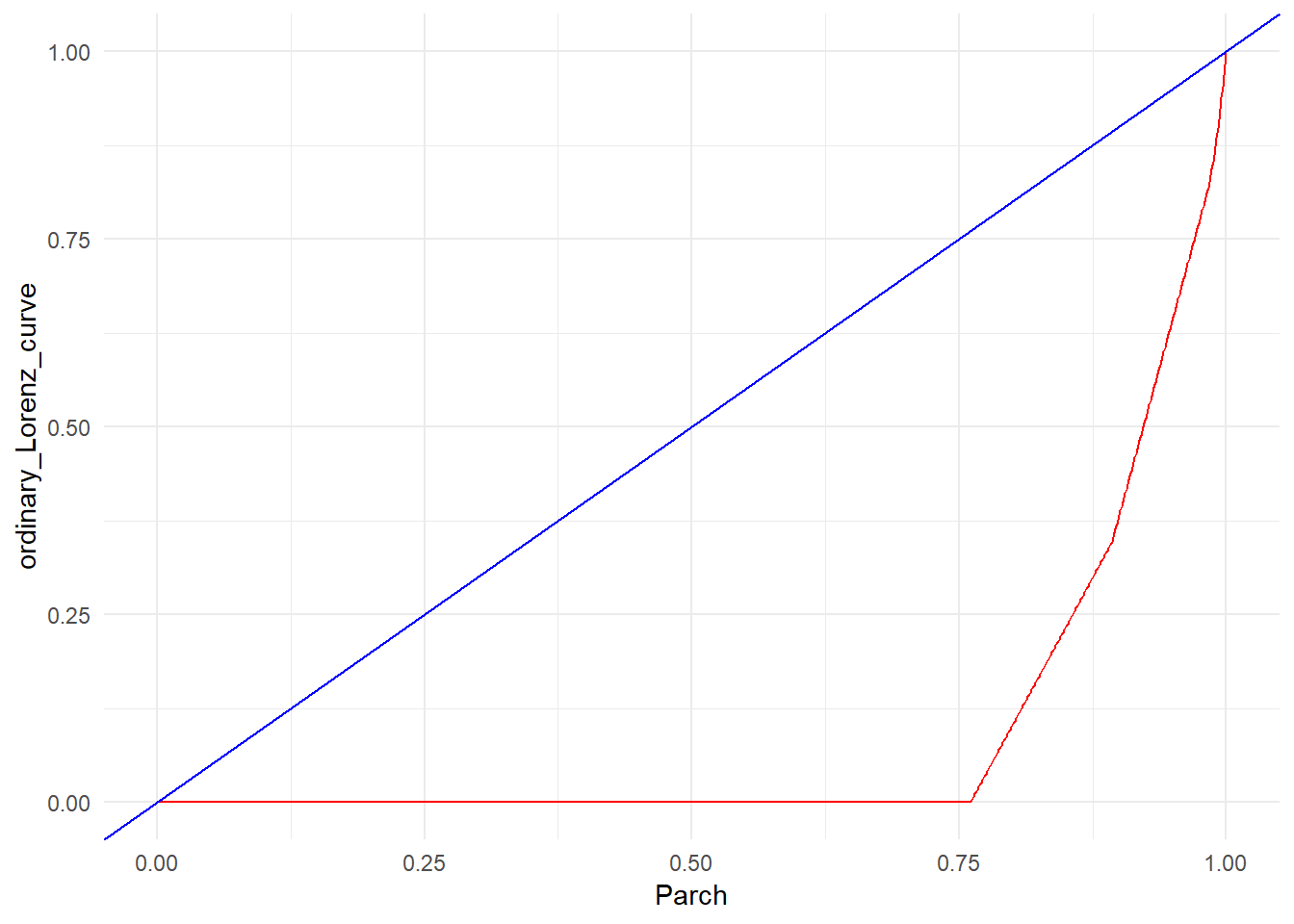##  "Gini coefficient = 0.818844703235625"
##  "----------------------------------------------------------------------------------------------------"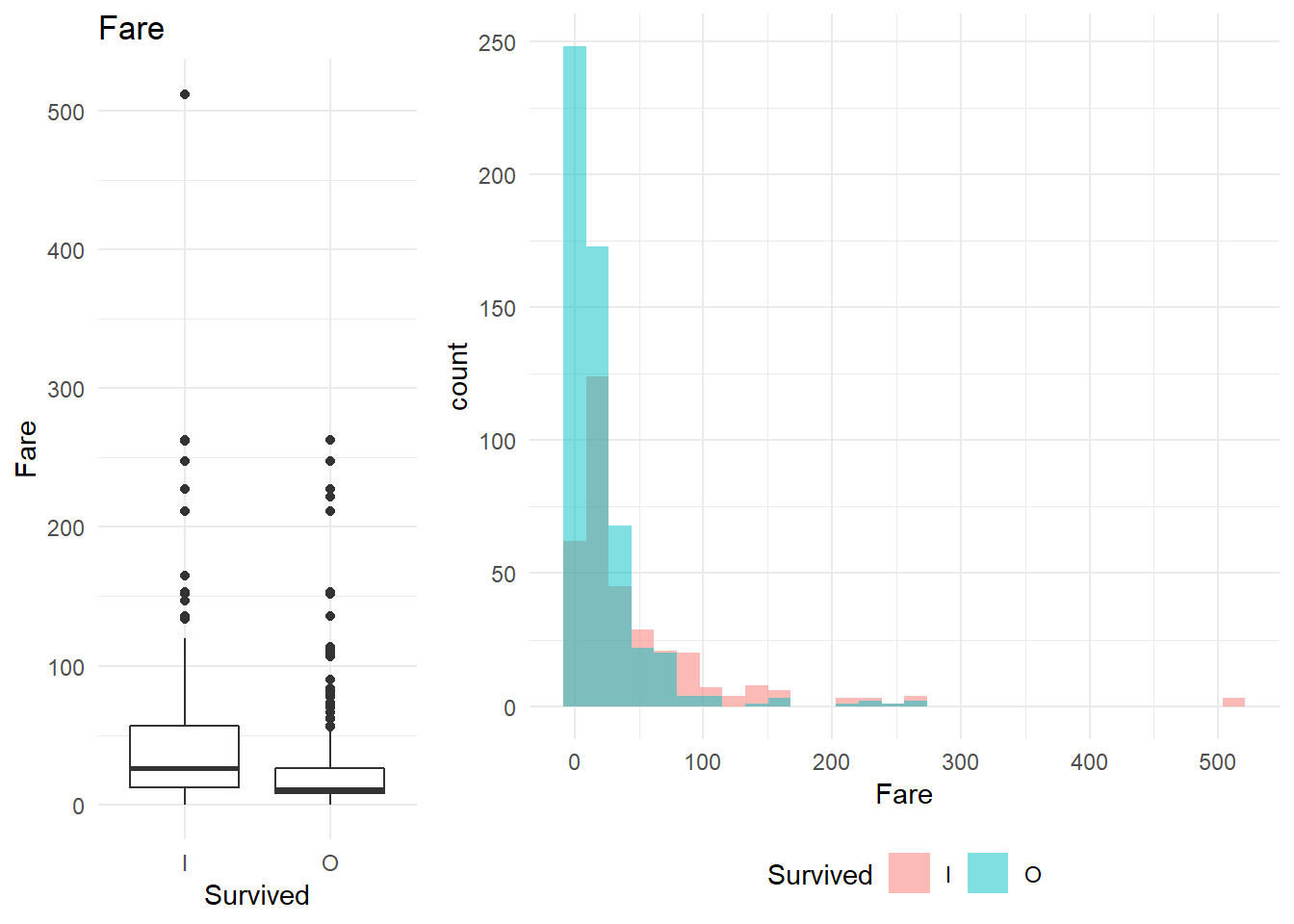##
## Call:
## NULL
##
## Deviance Residuals:
##     Min       1Q   Median       3Q      Max
## -1.5815  -1.3610   0.8594   0.8931   2.3627
##
## Coefficients:
##              Estimate Std. Error z value Pr(>|z|)
## (Intercept)  0.913285   0.105454   8.660  < 2e-16 ***
## Fare        -0.013845   0.002408  -5.749 8.95e-09 ***
## ---
## Signif. codes:  0 '***' 0.001 '**' 0.01 '*' 0.05 '.' 0.1 ' ' 1
##
## (Dispersion parameter for binomial family taken to be 1)
##
##     Null deviance: 947.02  on 711  degrees of freedom
## Residual deviance: 898.05  on 710  degrees of freedom
## AIC: 902.05
##
## Number of Fisher Scoring iterations: 4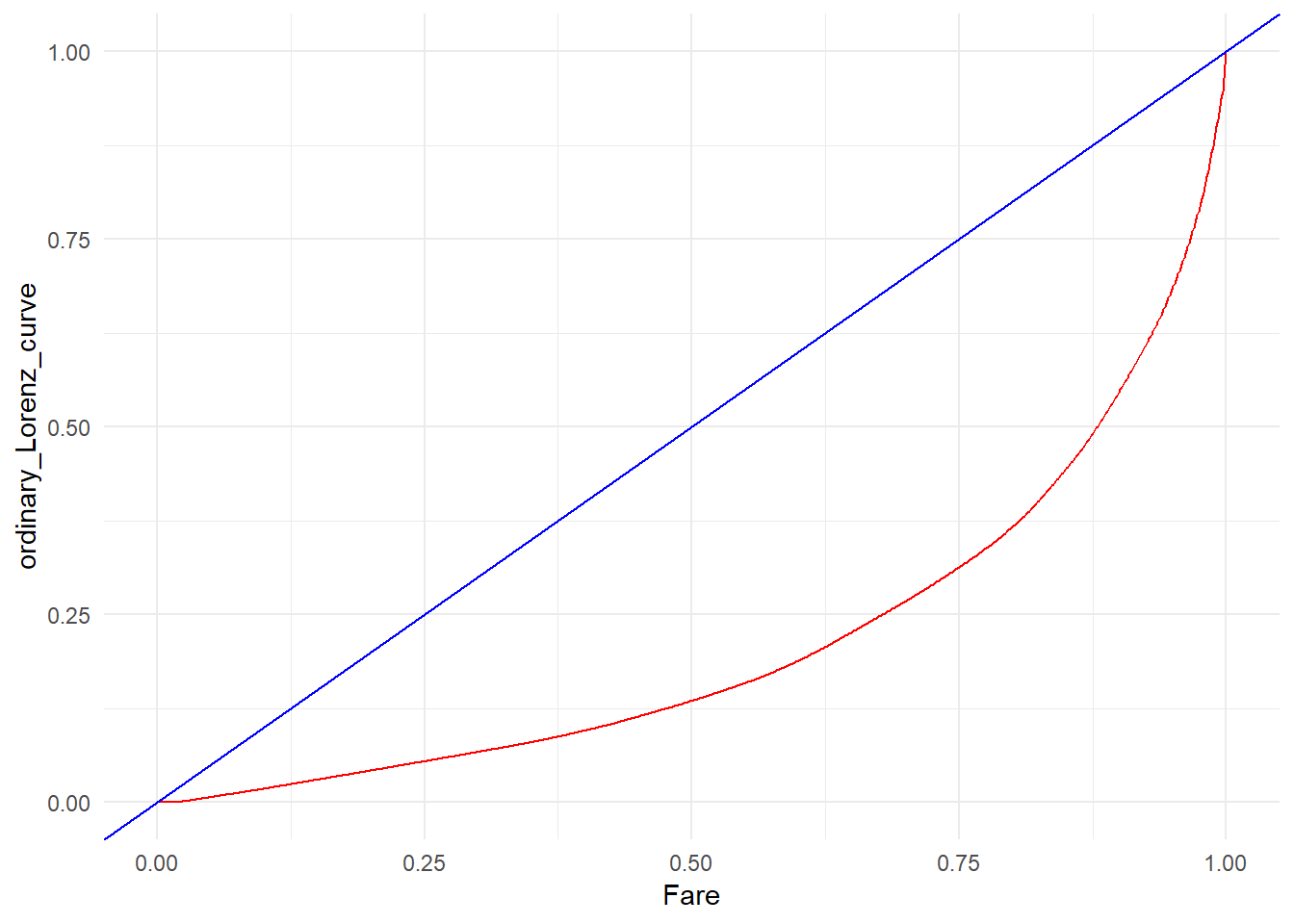##  "Gini coefficient = 0.570560662746992"
##  "----------------------------------------------------------------------------------------------------"

Observations:
1. From the box plot I observe that age and sibsp might not be significant factors. The same is reflected in the walds p value in the logistic regression. On the other hand, the gini coefficient is high for SibSp
2. Parch and Fare might be significant as I can observe a significant difference in the box plots.

I want to understand the relationship of each categorical variable with the $y$ variable. I will achieve that by doing the following:
1. A mosaic plot shows if any column is significantly different from base column
2. Predict using Logistic regression using the variable alone to observe the decrease in deviation/AIC

library(ggmosaic)
plot_bivariate_cat <- function(raw_d, pred_column_name){
plot_bi_cat_df <- raw_df %>% select_if(function(col) {is.factor(col) | is.character(col)})
for(column in colnames(plot_bi_cat_df)){
if(column != pred_column_name){
plot(ggplot(data = plot_bi_cat_df %>% group_by_(pred_column_name,column) %>% summarise(count = n())) +
geom_mosaic(aes_string(weight = 'count',
x = paste0("product(", pred_column_name," , ", column, ")"),
fill = pred_column_name), na.rm=TRUE) +
labs(x = column, y='%',  title = column) +
theme_minimal()+theme(legend.position="bottom") +
theme(axis.text.x=element_text(angle = 45, vjust = 0.5, hjust=1)))

trainList_cat <- createDataPartition(y = unlist(raw_df[pred_column_name]), times = 1,p = 0.8, list = FALSE)
dfTest_bi_cat <- raw_df[-trainList_cat,]
dfTrain_bi_cat <- raw_df[trainList_cat,]
form_2 = as.formula(paste0(pred_column_name,' ~ ',column))
set.seed(1234)
objControl <- trainControl(method = "none",
summaryFunction = twoClassSummary,
# sampling = 'smote',
classProbs = TRUE)

cat_loop_caret_model <- train(form_2, data = dfTrain_bi_cat,
method = 'glm',
trControl = objControl,
metric = "ROC")
print(summary(cat_loop_caret_model))
}
print(strrep("-",100))
}
}

plot_bivariate_cat(balanced_df, pred_column_name = 'Survived')
##  "----------------------------------------------------------------------------------------------------"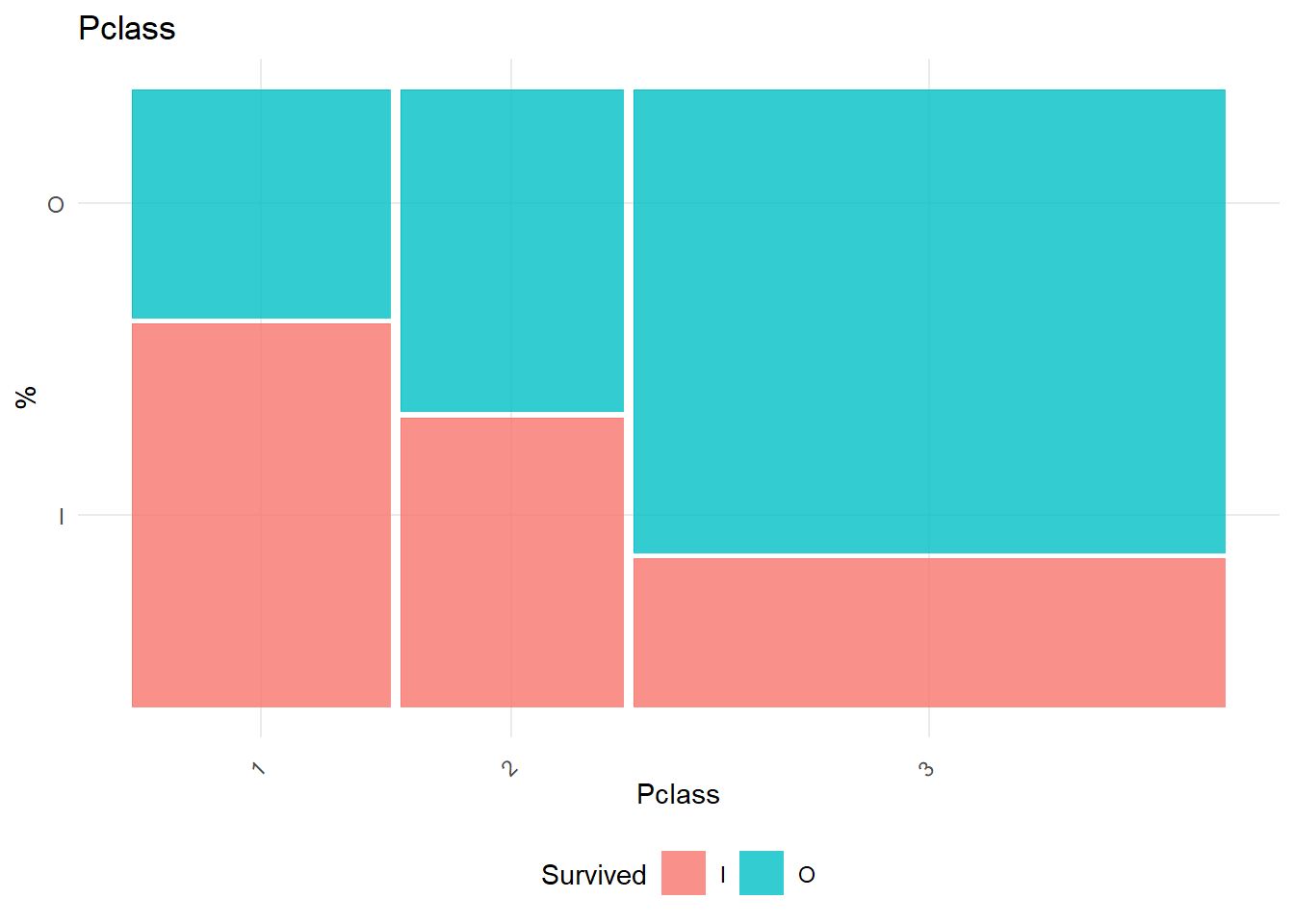##
## Call:
## NULL
##
## Deviance Residuals:
##     Min       1Q   Median       3Q      Max
## -1.6589  -0.9935   0.7631   0.7631   1.3732
##
## Coefficients:
##             Estimate Std. Error z value Pr(>|z|)
## (Intercept)  -0.4493     0.1564  -2.873  0.00406 **
## Pclass2       0.6340     0.2258   2.808  0.00499 **
## Pclass3       1.5342     0.1952   7.860 3.85e-15 ***
## ---
## Signif. codes:  0 '***' 0.001 '**' 0.01 '*' 0.05 '.' 0.1 ' ' 1
##
## (Dispersion parameter for binomial family taken to be 1)
##
##     Null deviance: 947.02  on 711  degrees of freedom
## Residual deviance: 877.96  on 709  degrees of freedom
## AIC: 883.96
##
## Number of Fisher Scoring iterations: 4
##
##  "----------------------------------------------------------------------------------------------------"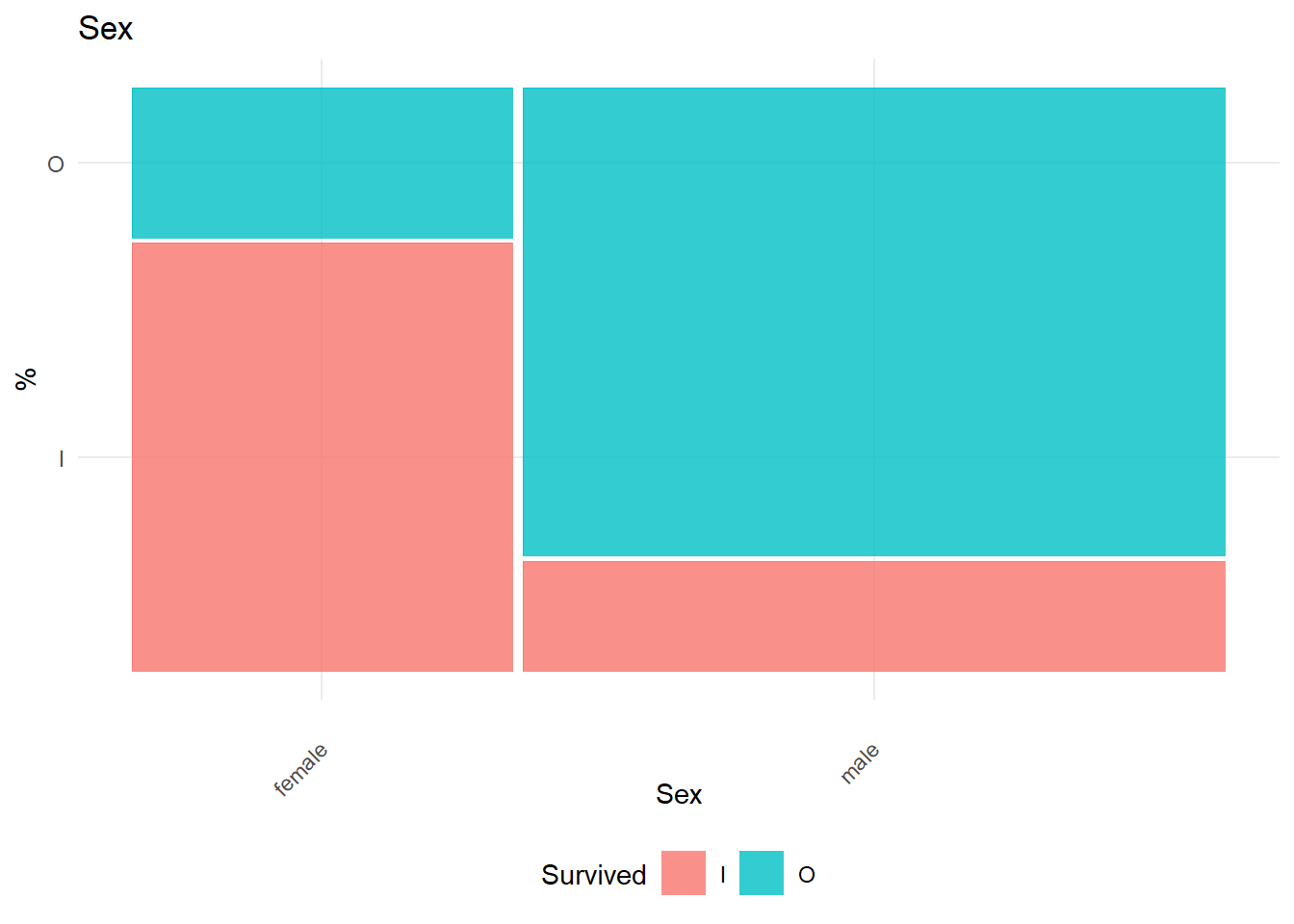##
## Call:
## NULL
##
## Deviance Residuals:
##     Min       1Q   Median       3Q      Max
## -1.8513  -0.7772   0.6304   0.6304   1.6398
##
## Coefficients:
##             Estimate Std. Error z value Pr(>|z|)
## (Intercept)  -1.0423     0.1421  -7.336  2.2e-13 ***
## Sexmale       2.5572     0.1873  13.656  < 2e-16 ***
## ---
## Signif. codes:  0 '***' 0.001 '**' 0.01 '*' 0.05 '.' 0.1 ' ' 1
##
## (Dispersion parameter for binomial family taken to be 1)
##
##     Null deviance: 947.02  on 711  degrees of freedom
## Residual deviance: 724.20  on 710  degrees of freedom
## AIC: 728.2
##
## Number of Fisher Scoring iterations: 4
##
##  "----------------------------------------------------------------------------------------------------"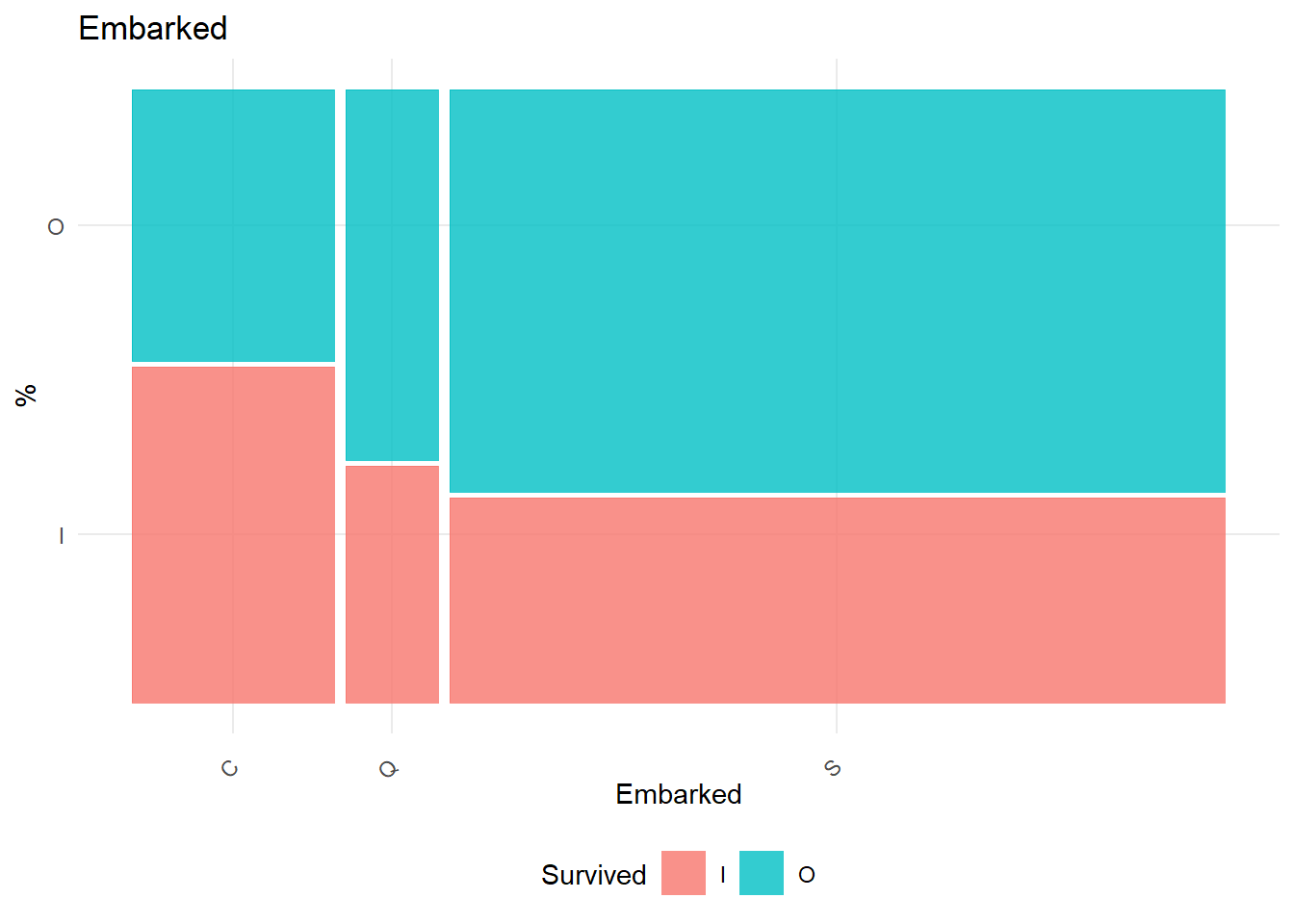##
## Call:
## NULL
##
## Deviance Residuals:
##     Min       1Q   Median       3Q      Max
## -1.4500  -1.4500   0.9274   0.9274   1.1961
##
## Coefficients:
##             Estimate Std. Error z value Pr(>|z|)
## (Intercept)  -0.0438     0.1709  -0.256 0.797729
## EmbarkedQ     0.5904     0.3178   1.858 0.063205 .
## EmbarkedS     0.6650     0.1943   3.422 0.000621 ***
## ---
## Signif. codes:  0 '***' 0.001 '**' 0.01 '*' 0.05 '.' 0.1 ' ' 1
##
## (Dispersion parameter for binomial family taken to be 1)
##
##     Null deviance: 947.02  on 711  degrees of freedom
## Residual deviance: 935.28  on 709  degrees of freedom
## AIC: 941.28
##
## Number of Fisher Scoring iterations: 4
##
##  "----------------------------------------------------------------------------------------------------"

Observations:
1. Gender seems to be a very important factor. The decrease in Residual due to that factor is very high.
2. There seems to be no significant difference between people embarked in Q vs C, but significant difference between C and S. From the plot, I could merge S and Q into one class for further analysis.
3. The class of the passenger seems to be an important factor.

### Correlation

The correlation between different variables is as follows

library(polycor)
corHet <- hetcor(as.data.frame(raw_df %>% mutate_if(is.character,as.factor)))
hetCorrPlot <- function(corHet){
melted_cormat <- reshape::melt(round(corHet,2), na.rm = TRUE)
colnames(melted_cormat) <- c('Var1', 'Var2', 'value')
melted_cormat <- melted_cormat %>% filter(!is.na(value))

# Plot the corelation matrix
ggheatmap <- ggplot(melted_cormat, aes(Var2, Var1, fill = value))+
geom_tile(color = "black")+
scale_fill_gradient2(low = "red", high = "green", mid = "white",
midpoint = 0, limit = c(-1,1), space = "Lab",
name="Heterogeneous Correlation Matrix") +
theme_minimal()+ # minimal theme
theme(axis.text.x = element_text(angle = 45, vjust = 1, size = 10, hjust = 1))+
geom_text(aes(Var2, Var1, label = value), color = "black", size = 4)+
theme(
axis.title.x = element_blank(),
axis.title.y = element_blank(),
panel.grid.major = element_blank(),
panel.border = element_blank(),
panel.background = element_blank(),
axis.ticks = element_blank()
)
print(ggheatmap)
}
hetCorrPlot(corHet$correlations)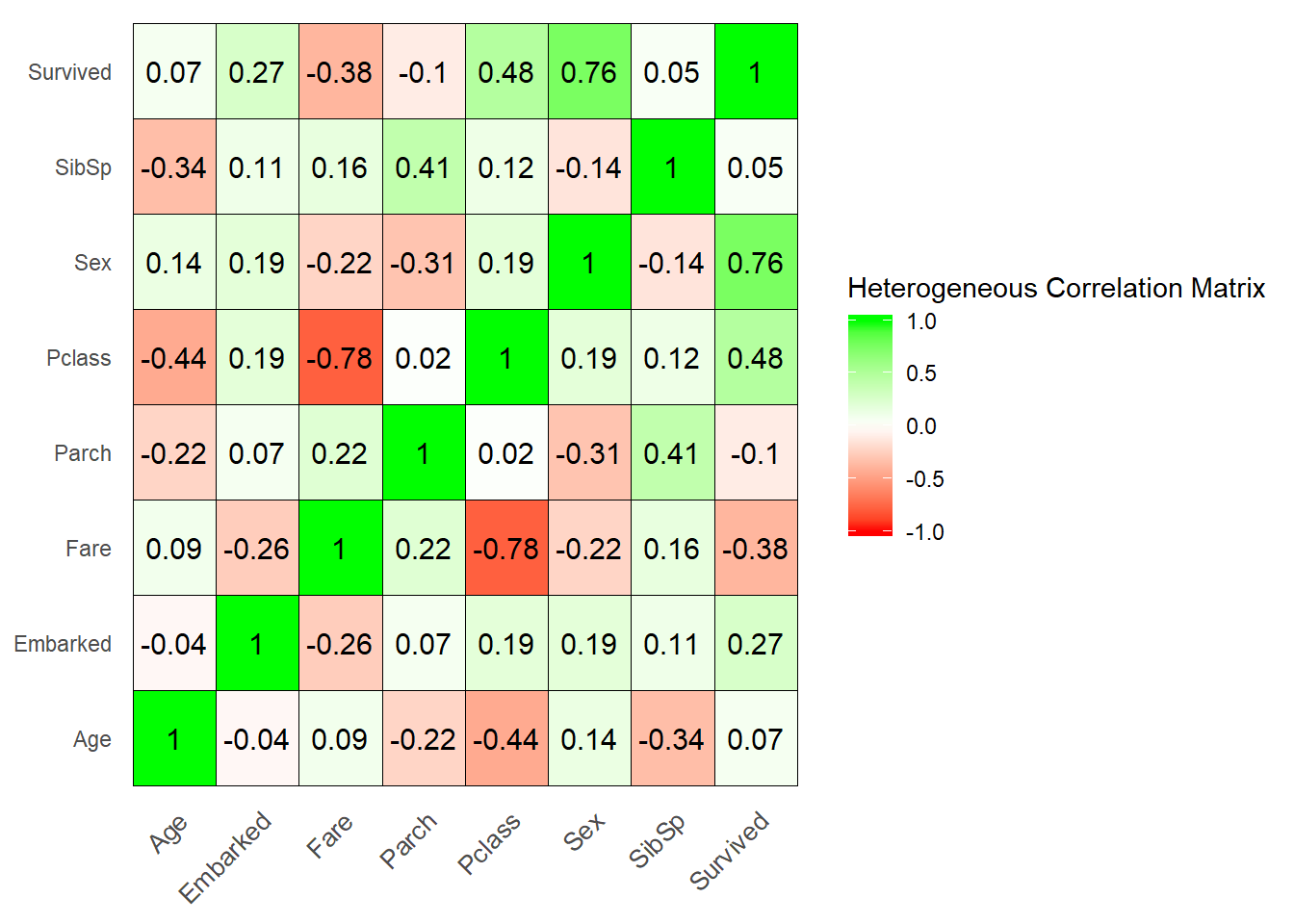Observations: 1. Passenger class and fare are negatively correlated (obvious). 2. Pclass and sex are two variables that have good correlation with the y variable(survived). ## Initial Model Training For my initial model, I am training using step wise logistic regression. In every step, I want to observe the following: 1. What variables are added or removed from the model. The current model pics the column which gives the greatest reduction in AIC. The model stops when the reduction in AIC w.r.t. null is lower than the threshold. 2. Substantial increase/decrease in $\beta$ or change in its sign (which may be due to colliniarity between the dependent variables) modified_df <- raw_df %>% mutate(Embarked = if_else(Embarked == 'C', 'C', 'S_or_Q')) trainList <- createDataPartition(y = modified_df$Survived, times = 1,p = 0.8, list = FALSE)
dfTest <- modified_df[-trainList,]
dfTrain <- modified_df[trainList,]
form_2 = as.formula(paste0('Survived ~ .'))
set.seed(1234)
objControl <- trainControl(method = "none",
summaryFunction = twoClassSummary,
classProbs = TRUE,
# sampling = 'smote',
savePredictions = TRUE)

model <- train(form_2, data = dfTrain,
method = 'glmStepAIC',
trControl = objControl,
metric = "ROC",
direction = 'forward'
)
## Start:  AIC=949.02
## .outcome ~ 1
##
##                  Df Deviance    AIC
## + Sexmale         1   724.20 728.20
## + Pclass3         1   885.94 889.94
## + Fare            1   898.05 902.05
## + EmbarkedS_or_Q  1   935.34 939.34
## + Age             1   940.79 944.79
## + Parch           1   941.94 945.94
## + Pclass2         1   942.85 946.85
## <none>                947.02 949.02
## + SibSp           1   946.05 950.05
##
## Step:  AIC=728.2
## .outcome ~ Sexmale
##
##                  Df Deviance    AIC
## + Pclass3         1   672.39 678.39
## + Fare            1   700.99 706.99
## + SibSp           1   710.98 716.98
## + EmbarkedS_or_Q  1   714.48 720.48
## + Parch           1   720.72 726.72
## + Pclass2         1   721.37 727.37
## <none>                724.20 728.20
## + Age             1   723.95 729.95
##
## Step:  AIC=678.39
## .outcome ~ Sexmale + Pclass3
##
##                  Df Deviance    AIC
## + Age             1   662.66 670.66
## + SibSp           1   664.21 672.21
## + Pclass2         1   664.36 672.36
## + EmbarkedS_or_Q  1   666.38 674.38
## + Fare            1   667.83 675.83
## <none>                672.39 678.39
## + Parch           1   670.60 678.60
##
## Step:  AIC=670.66
## .outcome ~ Sexmale + Pclass3 + Age
##
##                  Df Deviance    AIC
## + SibSp           1   646.62 656.62
## + Pclass2         1   648.58 658.58
## + EmbarkedS_or_Q  1   656.46 666.46
## + Parch           1   658.81 668.81
## + Fare            1   658.83 668.83
## <none>                662.66 670.66
##
## Step:  AIC=656.62
## .outcome ~ Sexmale + Pclass3 + Age + SibSp
##
##                  Df Deviance    AIC
## + Pclass2         1   629.48 641.48
## + Fare            1   638.87 650.87
## + EmbarkedS_or_Q  1   642.17 654.17
## <none>                646.62 656.62
## + Parch           1   646.28 658.28
##
## Step:  AIC=641.48
## .outcome ~ Sexmale + Pclass3 + Age + SibSp + Pclass2
##
##                  Df Deviance    AIC
## <none>                629.48 641.48
## + Fare            1   628.57 642.57
## + EmbarkedS_or_Q  1   628.68 642.68
## + Parch           1   628.98 642.98
print(summary(model))
##
## Call:
## NULL
##
## Deviance Residuals:
##     Min       1Q   Median       3Q      Max
## -2.5000  -0.6081   0.4231   0.6178   2.6988
##
## Coefficients:
##              Estimate Std. Error z value Pr(>|z|)
## (Intercept) -4.150857   0.464909  -8.928  < 2e-16 ***
## Sexmale      2.749566   0.217162  12.661  < 2e-16 ***
## Pclass3      2.285336   0.279783   8.168 3.13e-16 ***
## Age          0.044986   0.009111   4.938 7.91e-07 ***
## SibSp        0.445697   0.115171   3.870 0.000109 ***
## Pclass2      1.206182   0.297629   4.053 5.06e-05 ***
## ---
## Signif. codes:  0 '***' 0.001 '**' 0.01 '*' 0.05 '.' 0.1 ' ' 1
##
## (Dispersion parameter for binomial family taken to be 1)
##
##     Null deviance: 947.02  on 711  degrees of freedom
## Residual deviance: 629.48  on 706  degrees of freedom
## AIC: 641.48
##
## Number of Fisher Scoring iterations: 5

Observations:
1. Counter intuitively, both age and sibSp are significant features in the model while Parch and Fare are not. That might be due to Fare being explained by passenger class.
2. Gender, pclass are significant features while embarked is not

The model metrics for 50% cutoff are:

caretPredictedClass <- predict(object = model, dfTrain)
confusionMatrix(caretPredictedClass,dfTrain$Survived) ## Confusion Matrix and Statistics ## ## Reference ## Prediction I O ## I 192 62 ## O 80 378 ## ## Accuracy : 0.8006 ## 95% CI : (0.7693, 0.8293) ## No Information Rate : 0.618 ## P-Value [Acc > NIR] : <2e-16 ## ## Kappa : 0.5722 ## ## Mcnemar's Test P-Value : 0.1537 ## ## Sensitivity : 0.7059 ## Specificity : 0.8591 ## Pos Pred Value : 0.7559 ## Neg Pred Value : 0.8253 ## Prevalence : 0.3820 ## Detection Rate : 0.2697 ## Detection Prevalence : 0.3567 ## Balanced Accuracy : 0.7825 ## ## 'Positive' Class : I ##  ### Model Diagnostics The created model can be validated using various tests such as the Omnibus test, Wald’s test, Hosmer-Lemeshow’s test etc. Outliers can be validated thru residual plot, mahalanobis distance and dffit values, and finally I want to check for multicolliniarity and Pseudo R square. The Omnibus and Wald’s test have the following Null hypothesis $Omnibus\, H_0 : \beta_1 = \beta_2 = ... = \beta_k = 0$ $Omnibus\, H_1 : Not \, all\, \beta_i \,are\, 0$ And for each variable in the model $i$, $Wald's \, H_0 : \beta_i= 0$ $Wald's \, H_1 : \beta_i \neq 0$ Omnibus and Wald’s p values are given in the below table ## ## Call: ## NULL ## ## Deviance Residuals: ## Min 1Q Median 3Q Max ## -2.5000 -0.6081 0.4231 0.6178 2.6988 ## ## Coefficients: ## Estimate Std. Error z value Pr(>|z|) ## (Intercept) -4.150857 0.464909 -8.928 < 2e-16 *** ## Sexmale 2.749566 0.217162 12.661 < 2e-16 *** ## Pclass3 2.285336 0.279783 8.168 3.13e-16 *** ## Age 0.044986 0.009111 4.938 7.91e-07 *** ## SibSp 0.445697 0.115171 3.870 0.000109 *** ## Pclass2 1.206182 0.297629 4.053 5.06e-05 *** ## --- ## Signif. codes: 0 '***' 0.001 '**' 0.01 '*' 0.05 '.' 0.1 ' ' 1 ## ## (Dispersion parameter for binomial family taken to be 1) ## ## Null deviance: 947.02 on 711 degrees of freedom ## Residual deviance: 629.48 on 706 degrees of freedom ## AIC: 641.48 ## ## Number of Fisher Scoring iterations: 5 For all the factors for which p value is less than $\alpha=0.05$, I reject the Null hypothesis. These factors are significant factors for building the model. Hosmer Lemeshow test is a chi-square goodness of fit test to check if the logistic regression model fits the data. The Null hypothesis is that the model fits the data. library(ResourceSelection) dfTrain$pred_probability_I <- (predict(object = model, dfTrain,type = 'prob'))$I dfTrain$pred_probability_O <- (predict(object = model, dfTrain,type = 'prob'))$O dfTrain$predictions <- ifelse(dfTrain$pred_probability_I >= 0.5, 1, 0) dfTrain$binary <- ifelse(dfTrain$Survived == 'I', 1, 0) hoslem.test(dfTrain$predictions, dfTrain$binary, g = 10) ## ## Hosmer and Lemeshow goodness of fit (GOF) test ## ## data: dfTrain$predictions, dfTrain$binary ## X-squared = 1.9275, df = 8, p-value = 0.9832 Since the p-value is greater than $\alpha=0.05$ I accept the null hypothesis that the logistic regression fits the data. #### Checking for outliers: A simple residual plot can be useful to check outliers. library(arm) binnedplot(fitted(model$finalModel),
residuals(model$finalModel, type = "response"), nclass = NULL, xlab = "Expected Values", ylab = "Average residual", main = "Binned residual plot", cex.pts = 0.8, col.pts = 1, col.int = "gray")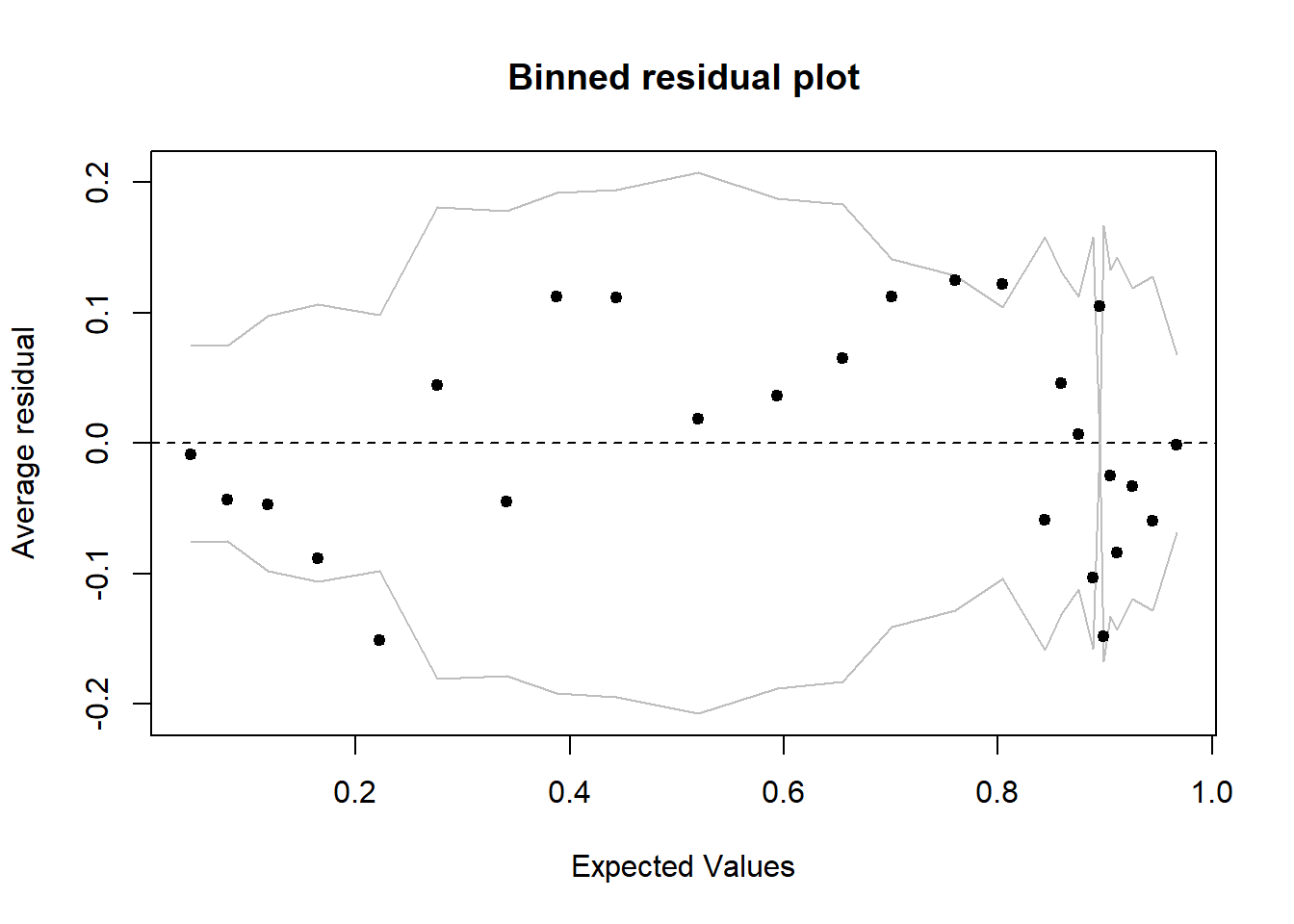The grey lines represent ± 2$\sigma$ bands, which we would expect to contain about 95% of the observations. This model does look reasonable as the majority of the fitted values seem to fall inside the SE bands and are randomly distributed. The mahalanobis distance gives the distance between the observation and the centroid of the values. An mahalanobis distance of greater than the chi-square critical value where the degrees of freedom is equal to number of independent variables is considered as an highly influential variable. maha.df <- dfTrain %>% dplyr::select(Age, SibSp) maha.df$maha <- mahalanobis(maha.df, colMeans(maha.df), cov(maha.df))
maha.df <- maha.df %>% arrange(-maha) %>% mutate(is.outlier = if_else(maha>12, TRUE, FALSE))
ggplot(maha.df, aes(y=Age, x= SibSp, color = is.outlier)) +
geom_point(show.legend = TRUE) +
theme_minimal()+theme(legend.position="bottom")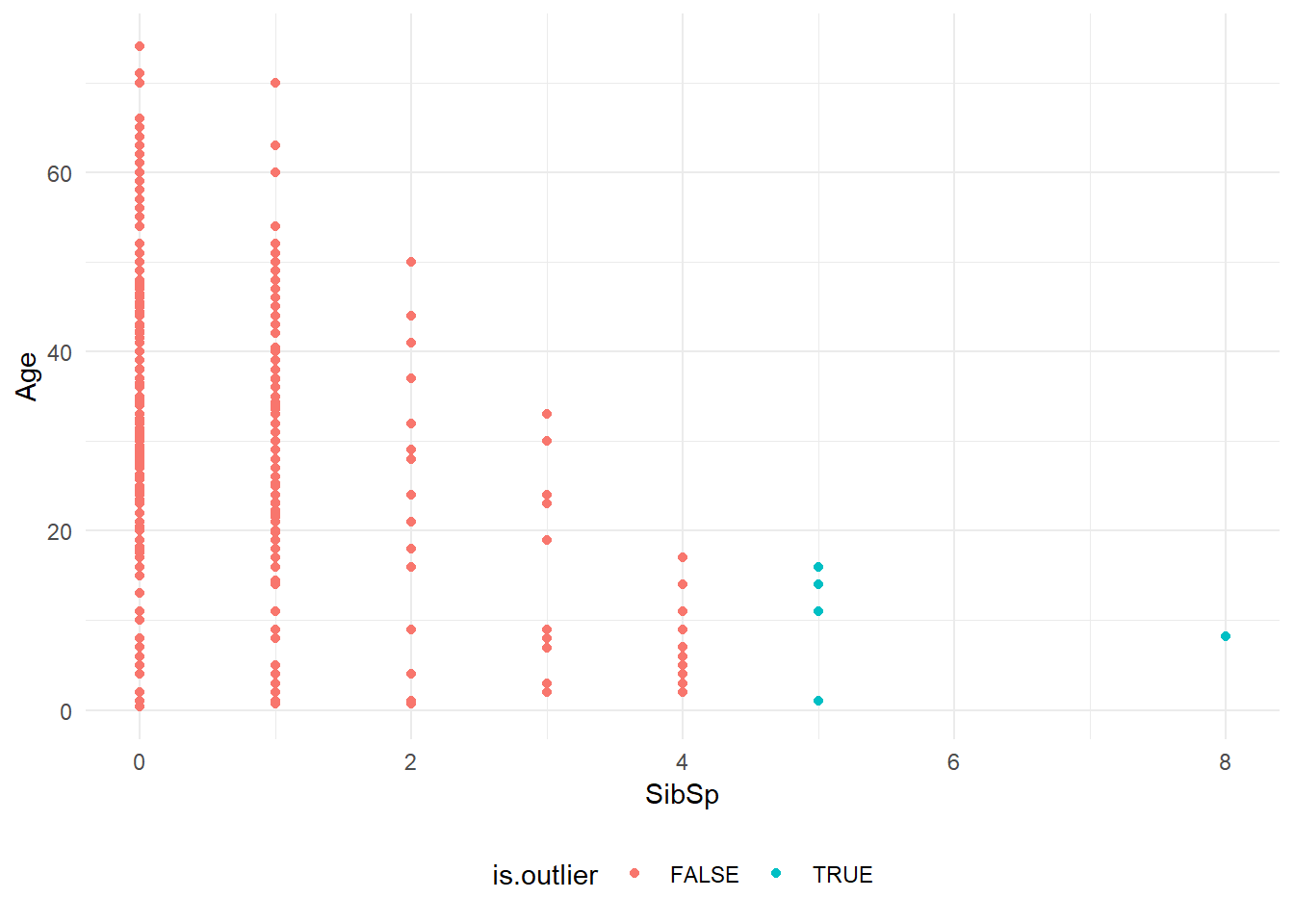Although the model predicts that certain observations are outliers, I am not doing any outlier treatment as they are observations that I am interested in.

#### Checking for multi collinearity

Like in case of linear regression, we should check for multi collinearity in the model. Multi collinearity is given by VIF. VIF above 4 means there is significant multicollinearity.

library(car)
vif(model$finalModel) ## Sexmale Pclass3 Age SibSp Pclass2 ## 1.147666 1.961434 1.446117 1.224168 1.630786 No factor has high multicollinearity(VIF>4). ### Finding optimal cutoff For finding the optimal cutoff, I am using three methods. 1. Classification plot 2. Youden’s Index 3. Cost based approach 1. Classification plot ggplot(dfTrain, aes(x = pred_probability_I, fill = Survived)) + geom_histogram(alpha=0.5, position="identity") + labs(x = 'Predicted probability', y='Count', title = 'Classification plot',fill = 'Observed Groups') + theme_minimal()+ theme(legend.position="bottom")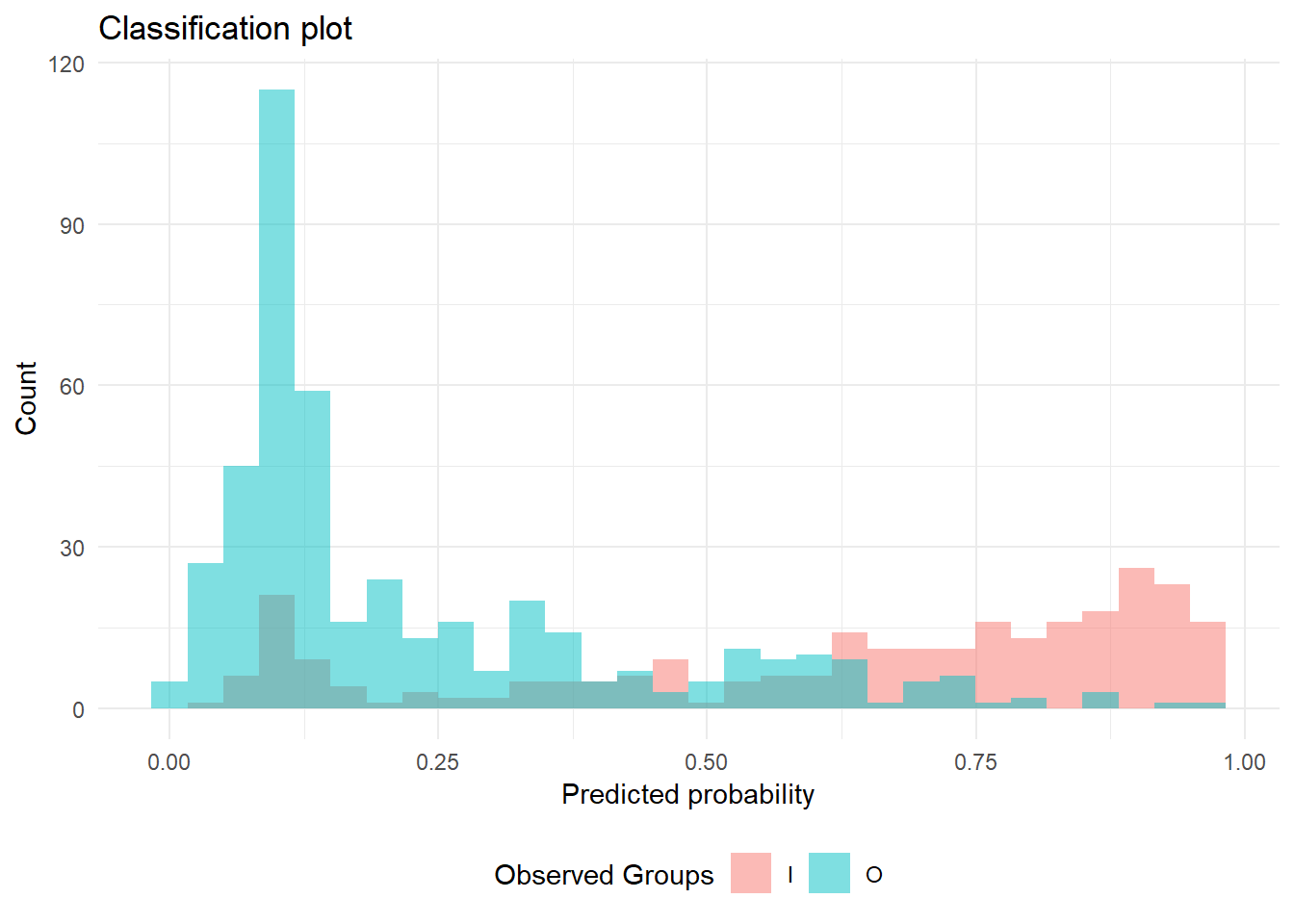1. Youden’s Index Youdens index can be used to find cutoff when sensitivity and specificity are equally important. It is the point which has minimum distance from ROC curve’s (1, 1) point. $Youden's\, Index \, = \, \max_{p} (Sensitivity(p),Specificity(p)-1)$ library(ROCR) lgPredObj <- prediction(dfTrain$pred_probability_I, dfTrain$Survived,label.ordering = c('O', 'I')) lgPerfObj <- performance(lgPredObj, "tpr", "fpr") plot(lgPerfObj, main = "ROC Curve (train data)", col = 2, lwd = 2) abline(a = 0, b = 1, lwd = 2, lty = 3, col = "black")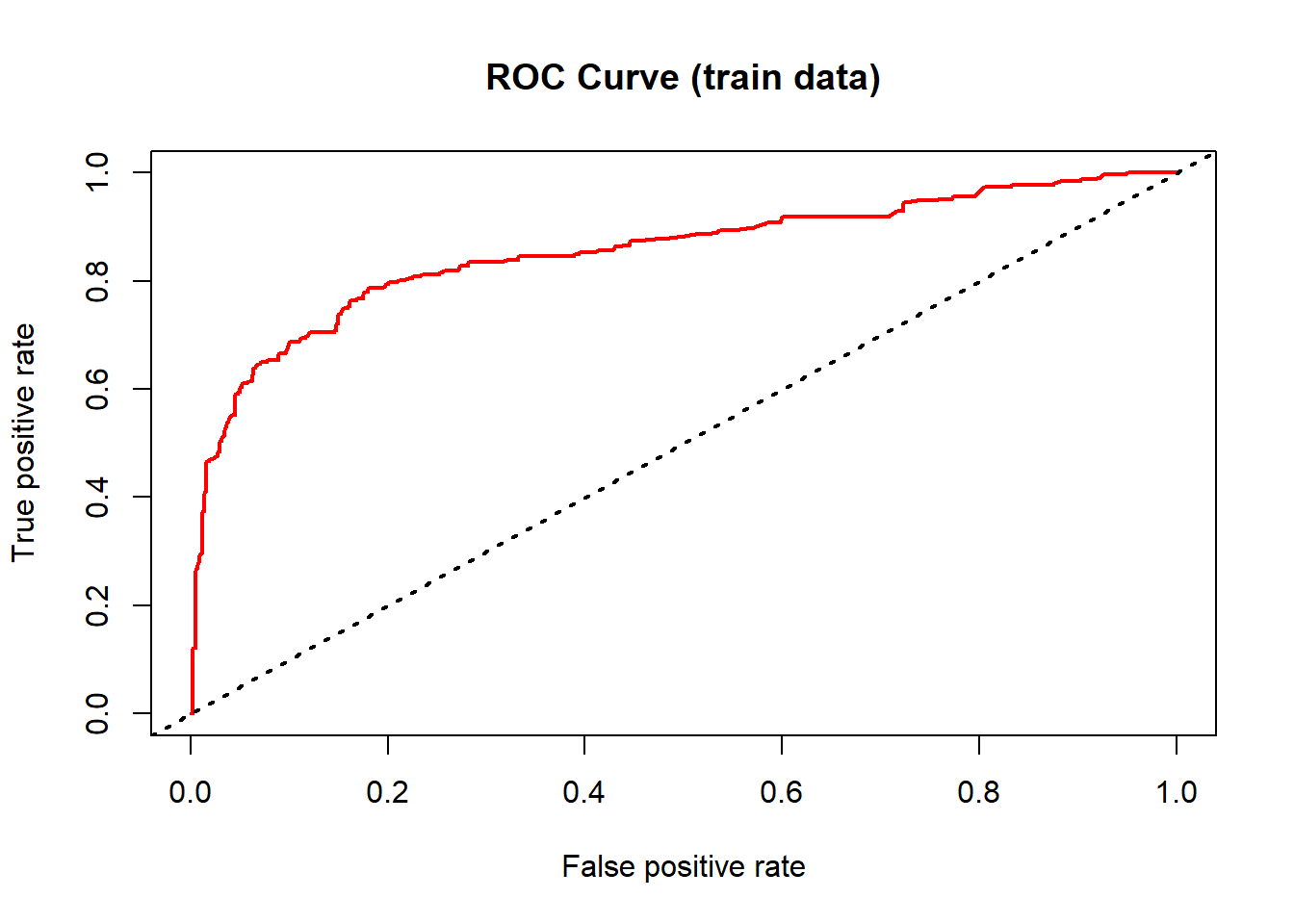It can also be visualized as the point where sensitivity and specificity are the same sens_spec_plot <- function(actual_value, positive_class_name, negitive_class_name, pred_probability){ # Initialising Variables specificity <- c() sensitivity <- c() cutoff <- c() for (i in 1:100) { predList <- as.factor(ifelse(pred_probability >= i/100, positive_class_name, negitive_class_name)) specificity[i] <- specificity(predList, actual_value) sensitivity[i] <- sensitivity(predList, actual_value) cutoff[i] <- i/100 } df.sens.spec <- as.data.frame(cbind(cutoff, specificity, sensitivity)) ggplot(df.sens.spec, aes(x = cutoff)) + geom_line(aes(y = specificity, color = 'Specificity')) + geom_line(aes(y = sensitivity, color = 'Sensitivity'))+ labs(x = 'Cutoff p value', y='Sens/Spec', title = 'Sensitivity-Specificity plot',fill = 'Plot') + theme_minimal()+ theme(legend.position="bottom") } sens_spec_plot(actual_value = dfTrain$Survived, positive_class_name = 'I', negitive_class_name = 'O', pred_probability = dfTrain$pred_probability_I)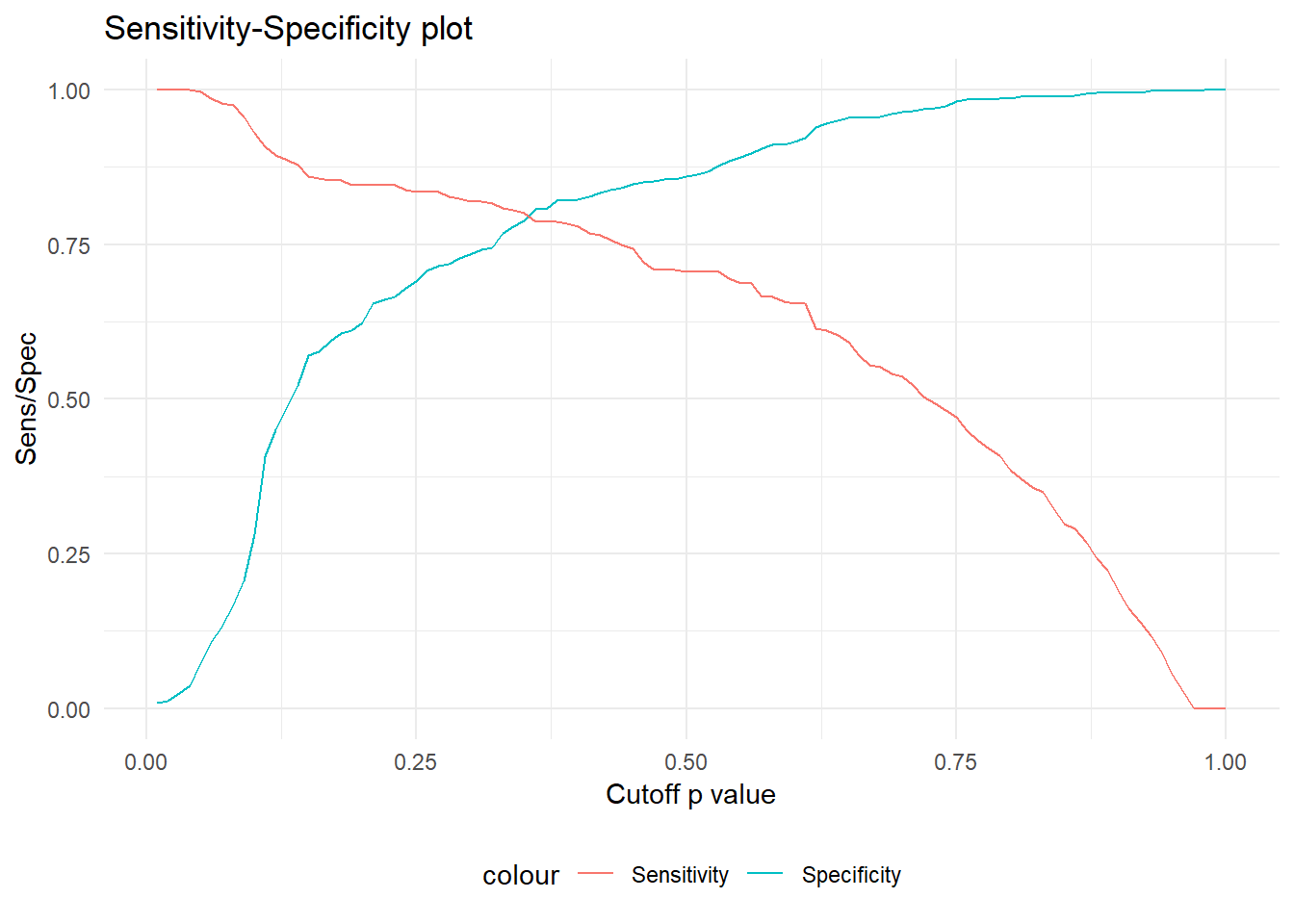1. Cost based approach I want to give penalties for positive and negatives. The optimal cutoff probability is the one which minimizes the total penalty cost, given by: $\min_p[C_{01}P_{01} + C_{10}P_{10}]$ For example, if I want to give 3 times the importance to predicting survived when compared to not survived, the cost table is: ## act I act O ## pred I 0 3 ## pred O 1 0 For both the above approaches, the cutoff is: find_p_cutoff <- function(actual_value, positive_class_name, negitive_class_name, pred_probability, p_01=1, p_10=1){ # Initialising Variables msclaf_cost <- c() youden_index <- c() cutoff <- c() P00 <- c() #correct classification of negative as negative (Sensitivity) P01 <- c() #misclassification of negative class to positive class (actual is 0, predicted 1) P10 <- c() #misclassification of positive class to negative class (actual 1 predicted 0) P11 <- c() #correct classification of positive as positive (Specificity) costs = matrix(c(0, p_01, p_10, 0), ncol = 2) for (i in 1:100) { predList <- as.factor(ifelse(pred_probability >= i/100, positive_class_name, negitive_class_name)) tbl <- table(predList, actual_value) # Classifying actual no as yes P00[i] <- tbl/(tbl + tbl) P01[i] <- tbl/(tbl + tbl) # Classifying actual yes as no P10[i] <- tbl/(tbl + tbl) P11[i] <- tbl/(tbl + tbl) cutoff[i] <- i/100 msclaf_cost[i] <- P10[i] * costs + P01[i] * costs youden_index[i] <- P11[i] + P00[i] - 1 } df.cost.table <- as.data.frame(cbind(cutoff, P10, P01, P11, P00, youden_index, msclaf_cost)) cat(paste0('The ideal cutoff for:\n Yodens Index approach : ', which.max(df.cost.table$youden_index)/100))
cat(paste0('\n Cost based approach : ', which.min(df.cost.table$msclaf_cost)/100)) ggplot(df.cost.table, aes(x = cutoff)) + geom_line(aes(y = youden_index, color = 'yoden index')) + geom_line(aes(y = msclaf_cost, color = 'misclassification cost'))+ labs(x = 'Cutoff p value', y='Index', title = 'Cutoff p value',fill = 'Plot') + theme_minimal()+ theme(legend.position="bottom") } find_p_cutoff(actual_value = dfTrain$Survived, positive_class_name = 'I', negitive_class_name = 'O', pred_probability = dfTrain$pred_probability_I, p_01 =3, p_10 = 1) ## The ideal cutoff for: ## Yodens Index approach : 0.38 ## Cost based approach : 0.27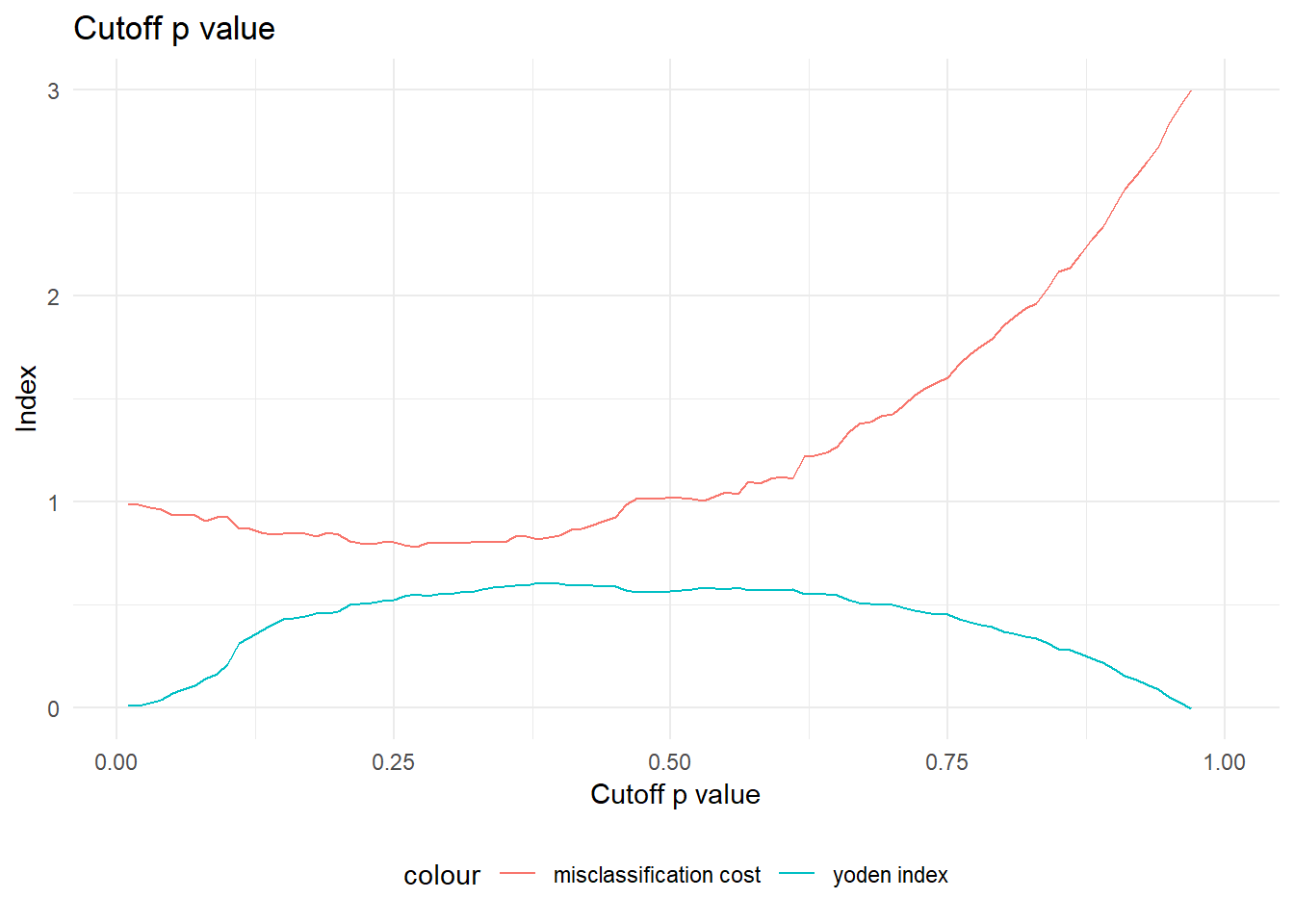I am going to consider a cutoff of 0.38. Final training model metrics: caretPredictedProb <- predict(object = model, dfTrain, type = 'prob') caretPredictedClass <- as.factor(ifelse(caretPredictedProb$I >= 0.38, 'I', 'O'))
confusionMatrix(caretPredictedClass,dfTrain$Survived) ## Confusion Matrix and Statistics ## ## Reference ## Prediction I O ## I 214 79 ## O 58 361 ## ## Accuracy : 0.8076 ## 95% CI : (0.7767, 0.8359) ## No Information Rate : 0.618 ## P-Value [Acc > NIR] : <2e-16 ## ## Kappa : 0.5984 ## ## Mcnemar's Test P-Value : 0.0875 ## ## Sensitivity : 0.7868 ## Specificity : 0.8205 ## Pos Pred Value : 0.7304 ## Neg Pred Value : 0.8616 ## Prevalence : 0.3820 ## Detection Rate : 0.3006 ## Detection Prevalence : 0.4115 ## Balanced Accuracy : 0.8036 ## ## 'Positive' Class : I ##  # AUC, ROC and other metrics summary_set <- data.frame(obs = dfTrain$Survived, pred = caretPredictedClass, I = caretPredictedProb$I) twoClassSummary(summary_set, lev = levels(summary_set$obs))
##       ROC      Sens      Spec
## 0.8538854 0.7867647 0.8204545
prSummary(summary_set, lev = levels(summary_set\$obs))
##       AUC Precision    Recall         F
## 0.8168413 0.7303754 0.7867647 0.7575221

## Validating the model on test data set

### Confusion matrix/specificity and sensitivity metrics

## Confusion Matrix and Statistics
##
##           Reference
## Prediction  I  O
##          I 45 18
##          O 23 91
##
##                Accuracy : 0.7684
##                  95% CI : (0.6992, 0.8284)
##     No Information Rate : 0.6158
##     P-Value [Acc > NIR] : 1.153e-05
##
##                   Kappa : 0.5036
##
##  Mcnemar's Test P-Value : 0.5322
##
##             Sensitivity : 0.6618
##             Specificity : 0.8349
##          Pos Pred Value : 0.7143
##          Neg Pred Value : 0.7982
##              Prevalence : 0.3842
##          Detection Rate : 0.2542
##    Detection Prevalence : 0.3559
##       Balanced Accuracy : 0.7483
##
##        'Positive' Class : I
## 

## ROC and lift charts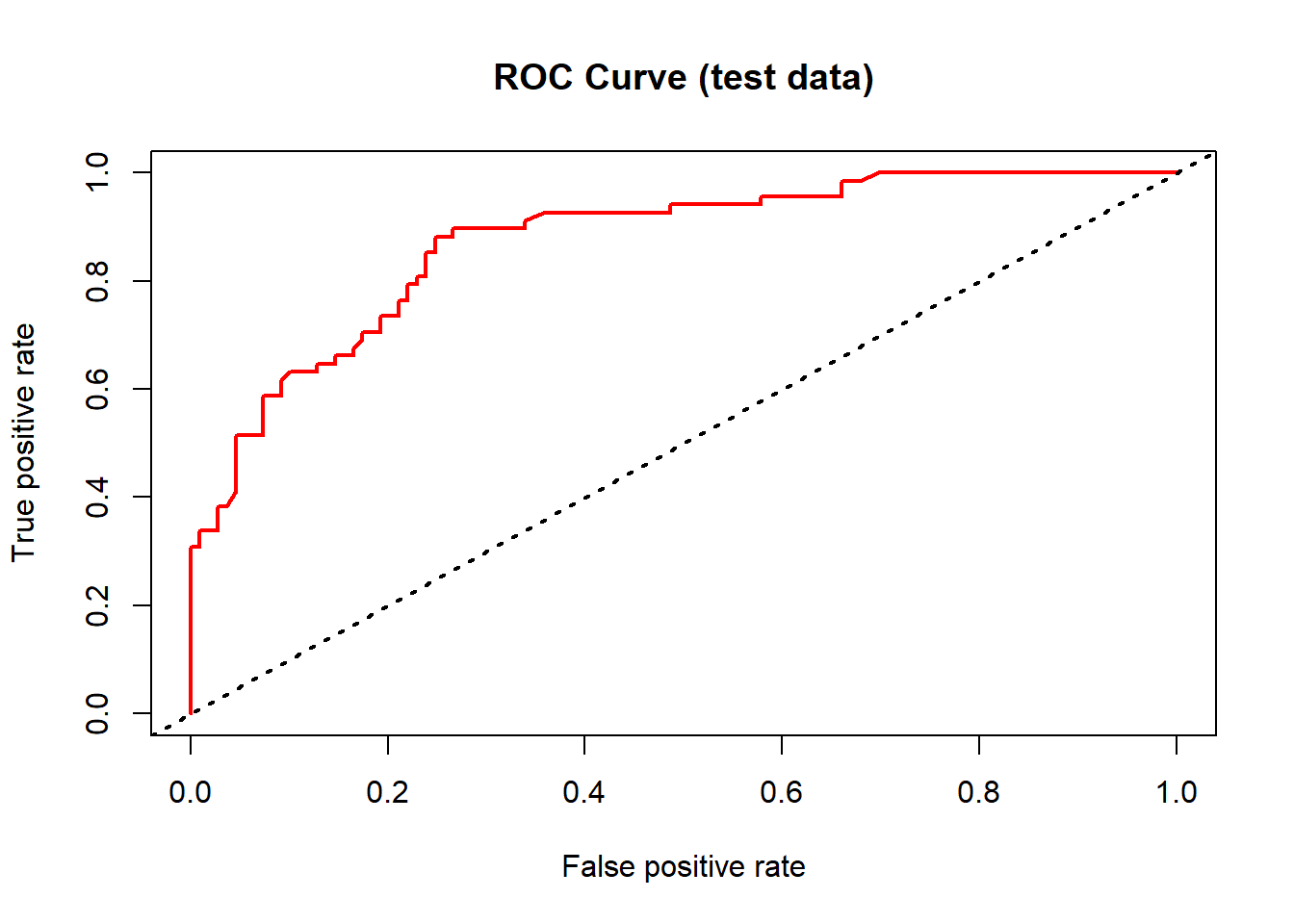roc_obj <- performance(lgPredObj, "lift", "rpp")
plot(roc_obj, main = "Lift chart (test data)", col = 2, lwd = 2)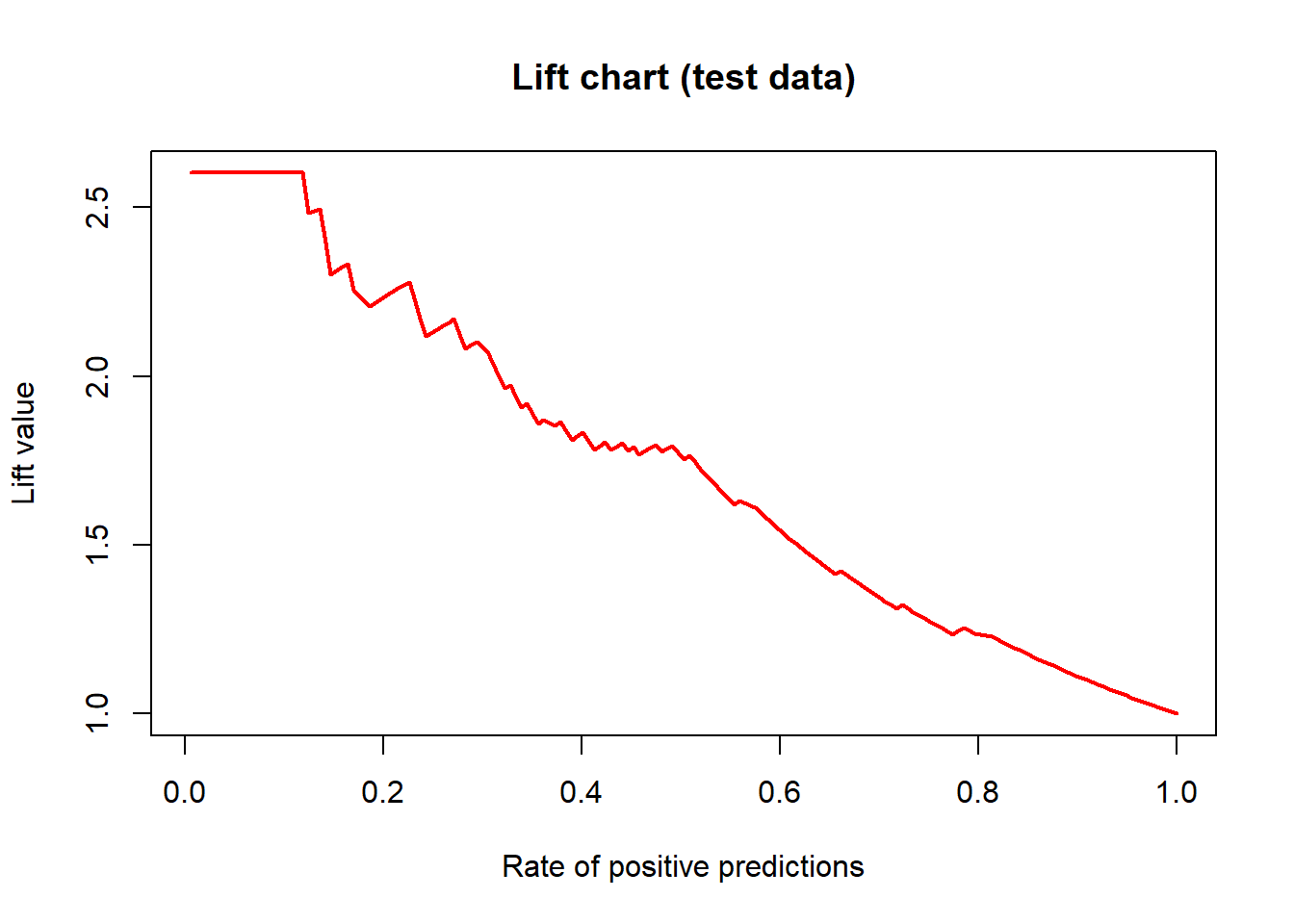trellis.par.set(caretTheme())
gain_obj <- lift(Survived ~ predictions, data = dfTrain)
ggplot(gain_obj) +
labs(title = 'Gain Chart') +
theme_minimal()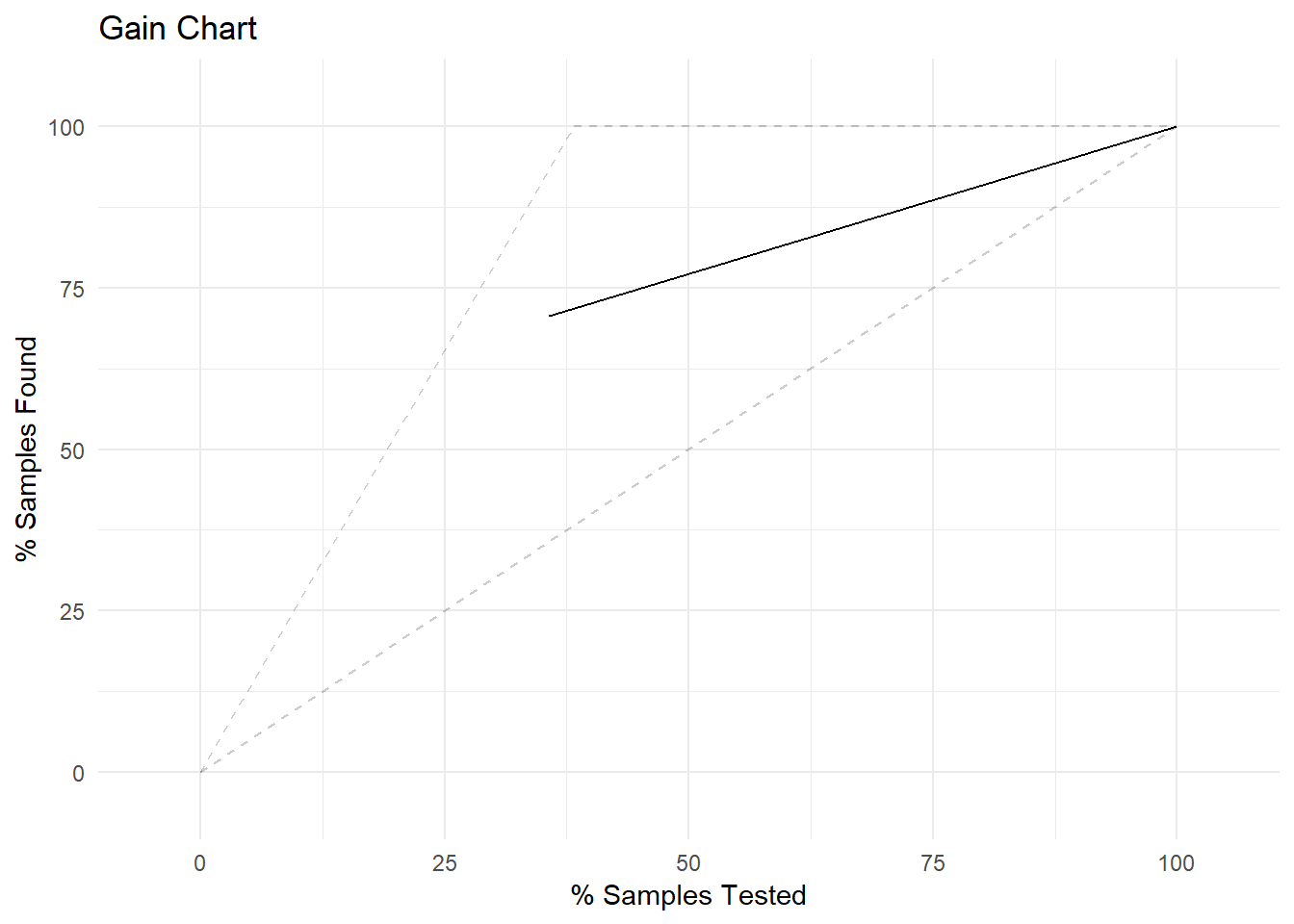The accuracy metrics on the test set are as follows:

##       ROC      Sens      Spec
## 0.8727739 0.6617647 0.8348624
##       AUC Precision    Recall         F
## 0.8114187 0.7142857 0.6617647 0.6870229

As our accuracy on the test set is similar to the accuracy on the training set and as all model validation checks are fine, I conclude that we can use Logistic regression to analyse what sort of people were likely to survive the titanic.

Summary:
Age, Passenger class and number of siblings are important metrics for the survival in titanic. Females, young people, people in higher class(proxy for rich people) and siblings had a higher chance of survival.

Tagged with: |